Wir verwenden Google für unsere Suche. Mit Klick auf „Suche aktivieren“ aktivieren Sie das Suchfeld und akzeptieren die Nutzungsbedingungen.

# New in SG-Lib 4.1

###### isonVLFLedge(VL,FL,PL)- returns the triangles a vertex is on edge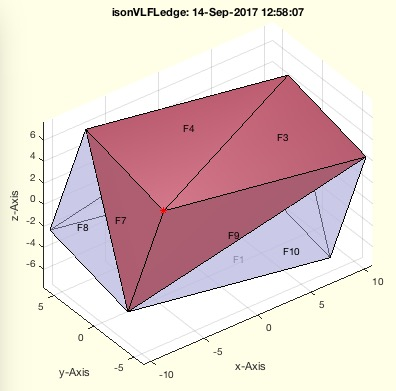% isonVLFLedge(VL,FL,PL) - returns the triangles a vertex is on edge% (by Tim Lueth, VLFL-Lib, 2017-SEP-14 as class: SURFACES)% % SGintersectFacetPoints returns a vertex list of crossing points of two % surfaces. Nevertheless in case of numerical problems when a vertex is % on a a facet edge, it is not found on the corresponding negative edge % of the neighbor facet. Therefor this fnctn just makes a cross check for % all vertices and all points% NN cols; [vi fi ei ti]% vi = checked vertex index of PL% fi = facets of FL that contains PL(vi,:)% ei = edge index 1..3 of fi% ti = value between 0 and 1 end point% if ti<0 or ti>1, the point is not on the edge (Status of: 2017-09-14)% % Introduced first in SolidGeometry 4.1% % See also: SGintersectFacetPoints, VLFLinsertFacetPoints% % NN=isonVLFLedge(VL,FL,PL)% === INPUT PARAMETERS ===% VL: Vertex list of solid% FL: Face list of solid% PL: Point to check or Point List% === OUTPUT RESULTS ======% NN: Result [n x 4]% % EXAMPLE: % SGsample(26); A=ans, isonVLFLedge(A.VL,A.FL,[0 -10 5])% SGsample(26); A=ans, isonVLFLedge(A.VL,A.FL,[0 -10 5;10 10 -5])% SGsample(26); A=ans, isonVLFLedge(A.VL,A.FL,[0 -10 5;20 10 -5])% % See also: SGintersectFacetPoints, VLFLinsertFacetPoints% % % Copyright 2017 Tim C. Lueth
###### barytocartUVLdelaunay(p1,p2,p3,UVL)- converts a 2d Barycentric [u,v] vertex list into a 3D Cartesian coordinate list [x, y, z]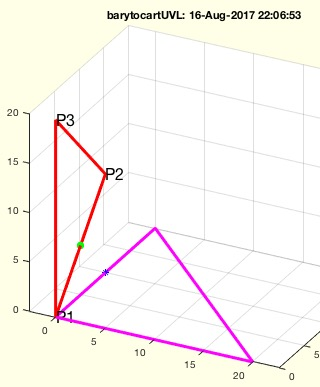% barytocartUVLdelaunay(p1,p2,p3,UVL) - converts a 2d Barycentric [u,v] vertex list into a 3D Cartesian coordinate list [x, y, z]% (by Tim Lueth, VLFL-Lib, 2017-SEP-11 as class: ANALYTICAL GEOMETRY)% % Same as barytocartUVL but uses mathlabs triangulation concept% Expecting all vertices in the plane of a triangle p1p2p3, the % coordinates of the vertices can be formulated as p1 + u*(p2-p1) + v* % (p3-p1). The Barycentric coordinates are [u v].% Both procedures can be used to solve 3D problems in 2D% % barytocartUVL is unable to detect the distance to the plane!% (Status of: 2017-09-11)% % Introduced first in SolidGeometry 4.1% % See also: carttobaryVL, barytocartUVL, clipbarycentric% % VL=barytocartUVLdelaunay(p1,p2,p3,UVL)% === INPUT PARAMETERS ===% p1: Point 1 of the triangle% p2: Point 2 of the triangle% p3: Point 3 of the triangle% UVL: Barycentric vertex list, all points in plane% === OUTPUT RESULTS ======% VL: n x 3 list with [x y z]% % EXAMPLE: % barytocartUVLdelaunay([0 0 0],[ 0 10 10],[0 0 20],[0 0.5])% % See also: carttobaryVL, barytocartUVL, clipbarycentric% % % Copyright 2017 Tim C. Lueth
###### ELofELmesh(EL)- returns a separated EL consisting of independent meshes and a rest of points and lines% ELofELmesh(EL) - returns a separated EL consisting of independent meshes and a rest of points and lines% (by Tim Lueth, VLFL-Lib, 2017-SEP-10 as class: EDGE LISTS)% % This fnctn will take an edge list and searches for closed meshed under % the condition of correct edge lists(!), which is not the case for % feature edges.% The results are closed meshes and a list of open lines and points % (Status of: 2018-08-28)% % Introduced first in SolidGeometry 4.1% % See also: ELplot, graphofEL% % [NEL,CIL,ELu]=ELofELmesh(EL)% === INPUT PARAMETERS ===% EL: Original edge list% === OUTPUT RESULTS ======% NEL: New edge list (blue)% CIL: CIL of meshes% ELu: list of restpoints that are not part of a mesh (red)% % EXAMPLE: % ELofELmesh([1 2;2 3;3 1;4 1])% ELofELmesh([1 2;2 3;1 3])% % See also: ELplot, graphofEL% % % Copyright 2017-2018 Tim C. Lueth
###### ELplot(EL)- plots the graph of the given edge list% ELplot(EL) - plots the graph of the given edge list% (by Tim Lueth, FileMaker, 2017-SEP-09 as class: EDGE LISTS)% % This fnctn uses the digraph class and plot for drawing the edge list.% (Status of: 2018-04-18)% % Introduced first in SolidGeometry 4.1% % See also: graphofEL% % [h,G]=ELplot(EL)% === INPUT PARAMETERS ===% EL: Edge List% === OUTPUT RESULTS ======% h: handle to plot% G: digraaph of the edge list% % EXAMPLE: % ELplot(FEofSG(SGsample(32)));% CPL=[PLcircle(5);nan nan;PLcircle(3)]; [~,EL]=PLELofCPL(CPL)% SGfigure; ELplot(EL);% X=SGsample(26); EL=ELofFL(X.FL); SGfigure; ELplot(EL,'layout','force3')% % See also: graphofEL% % % Copyright 2017-2018 Tim C. Lueth
###### ELconnectedgroups(EL,vi)- returns edge lists in groups of isolate indices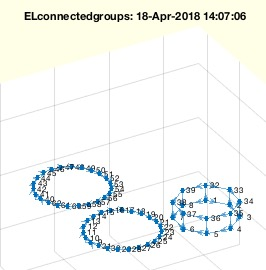% ELconnectedgroups(EL,vi) - returns edge lists in groups of isolate indices% (by Tim Lueth, VLFL-Lib, 2017-SEP-08 as class: EDGE LISTS)% % Use CILofEL afterwards% Use CELofEL to create EL with same directions (Status of: 2018-08-24)% % Introduced first in SolidGeometry 4.1% % See also: CELofEL, ELreconnect, ELofELmesh% % [NEL,CIL]=ELconnectedgroups(EL,vi)% === INPUT PARAMETERS ===% EL: Edge list% vi: Optional list of interested vertices; default is ''% === OUTPUT RESULTS ======% NEL: New Edge list; NOT SORTED ONLY GROUPED% CIL: Group Index List% % EXAMPLE: % ELconnectedgroups(FEofSG(SGsample(32)))% [NEL,CIL]=ELconnectedgroups( floor(rand(10,2)*10+1))% % See also: CELofEL, ELreconnect, ELofELmesh% % % Copyright 2017-2018 Tim C. Lueth
###### debugTL()- just prompts the current function as debug info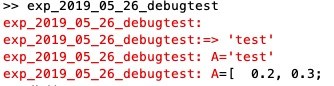% debugTL() - just prompts the current fnctn as debug info% (by Tim Lueth, VLFL-Lib, 2017-SEP-06 as class: AUXILIARY PROCEDURES)% % the varargin parameter has no use but the possibility to integrate % somehow the date of the debug process somehow in the code, even it is % useful only for a human programmer (Status of: 2017-09-06)% % Introduced first in SolidGeometry 4.1% % debugTL([])% % EXAMPLE: debugTL('2017-09-07');% % % Copyright 2017 Tim C. Lueth
###### CVLofSGcutTrot(SG,T,wlim,grow)- creates contours and 2.5D solids from a rotated cross-slice of a solid.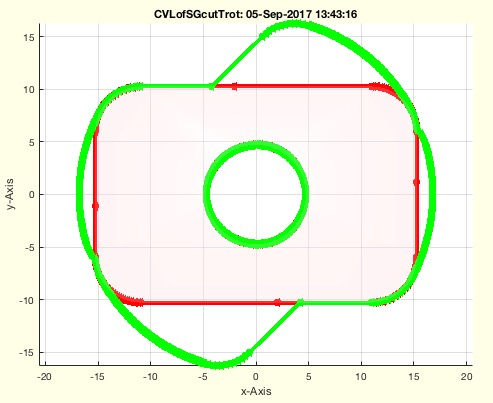% CVLofSGcutTrot(SG,T,wlim,grow) - creates contours and 2.5D solids from a rotated cross-slice of a solid. % (by Tim Lueth, VLFL-Lib, 2017-SEP-05 as class: SLICES)% % uses CVLofSGT but creates the sweeped crossing area and a 2D.% This fnctn is required if an rotating solid needs volume space for the % rotation and the revolving solid has to be substracted from the % rotation partner. (Status of: 2017-09-06)% % Introduced first in SolidGeometry 4.1% % See also: SGbool% % [CVLA,CVLB,CPLA,CPLB,A,B]=CVLofSGcutTrot(SG,[T,wlim,grow])% === INPUT PARAMETERS ===% SG: Solid Geoemtry% T: Cutting Frame; default is eye(4)% wlim: [wlim wmax]; default is [-pi/4 +pi/4];% % grow: growing factor / slot size% === OUTPUT RESULTS ======% CVLA: Spatial contour [n x 3] of above line% CVLB: Spatial contour [n x 3] of below line% CPLA: Planar contour [n x 2] of above line% CPLB: Planar contour [n x 2] of below line% A: Solid for contour A% B: Solid for contour B% % EXAMPLE: % CVLofSGcutTrot(SGsample(26),TofT(eye(4),0,+4),[0 pi/4]);% CVLofSGcutTrot(SGsample(27),TofT(eye(4),0,+4),[0 pi/4]);% % See also: SGbool% % % Copyright 2017 Tim C. Lueth
###### SGvertexongrid(A,grid1,grid2)- returns a solid geometry with all vertices set to a grid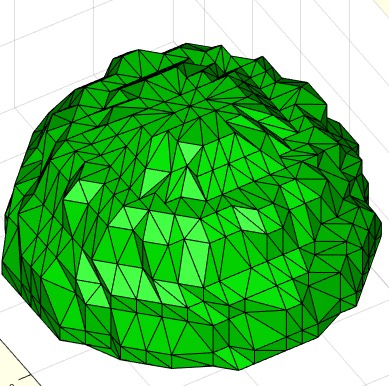% SGvertexongrid(A,grid1,grid2) - returns a solid geometry with all vertices set to a grid% (by Tim Lueth, VLFL-Lib, 2017-SEP-01 as class: SURFACES)% % Three important fnctns to be used in combination with SGbool:% SGvertexongrid - maps all vertices of a solid to a grid% SGretesselate - removes small facets from a solid% SGdelaunay - retesselates alls surfaces by delaunay% (Status of: 2017-09-07)% % Introduced first in SolidGeometry 4.1% % See also: VLFLvertexongrid, SGretesselate, SGdelaunay% % B=SGvertexongrid([A,grid1,grid2])% === INPUT PARAMETERS ===% A: Solid Geoemtry% grid1: default is 1e-3 points mapped to the same coordinates% grid2: default is 0.25*grid 1 grid the last point is mapped to% === OUTPUT RESULTS ======% B: Final Solid% % EXAMPLE: % SG=SGsample(5), SGvertexongrid(SG,1), VLmindxyz(ans)% % See also: VLFLvertexongrid, SGretesselate, SGdelaunay% % % Copyright 2017 Tim C. Lueth
###### VLFLvertexongrid(VL,FL,grid1,grid2)- returns a solid geometry with all vertices set to a grid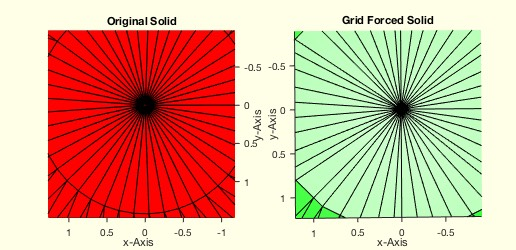% VLFLvertexongrid(VL,FL,grid1,grid2) - returns a solid geometry with all vertices set to a grid% (by Tim Lueth, VLFL-Lib, 2017-SEP-01 as class: SURFACES)% % Important fnctn to be used in combination with SGbool% This fnctn uses two grids.% grid 1 is used to select all points that are conidered to have the same % coordinates% grid 2 is used to force the remaining single point to a grid position% grid 2 is an order of magnitude smaller than grid 1 (Status of: % 2017-09-01)% % Introduced first in SolidGeometry 4.1% % See also: SGbool, VLFLremoveVertex% % [VLN,FLN]=VLFLvertexongrid([VL,FL,grid1,grid2])% === INPUT PARAMETERS ===% VL: Vertex List or Solid Geoemtry% FL: Facet List, or empty if VL=SG% grid1: default is 1e-3% grid2: default is 0.25*grid 1% === OUTPUT RESULTS ======% VLN: Final Vertex List or Final Solid if nargout==1% FLN: Final Facet List% % EXAMPLE: % A=SGsample(5), B=VLFLvertexongrid(A,'',0.1), VLmindxyz(B)% % See also: SGbool, VLFLremoveVertex% % % Copyright 2017 Tim C. Lueth
###### uniquehist(A,)- performs the unique command and creates a full histogram count afterwards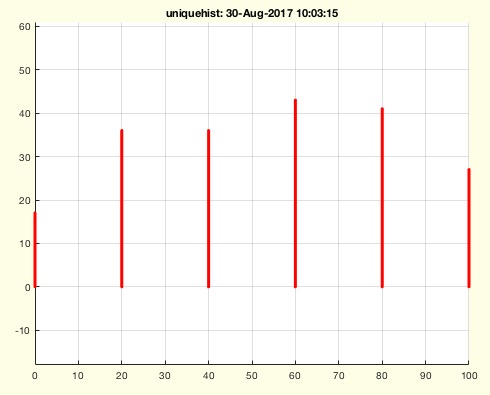% uniquehist(A,) - performs the unique command and creates a full histogram count afterwards% (by Tim Lueth, VLFL-Lib, 2017-AUG-30 as class: AUXILIARY PROCEDURES)% % works exactly as unique but calculates the number of occurences % afterwards (Status of: 2017-08-30)% % Introduced first in SolidGeometry 4.1% % See also: unique% % [C,ia,ic,h]=uniquehist(A,[])% === INPUT PARAMETERS ===% A: array A% === OUTPUT RESULTS ======% C: unique arrary, may be sorted% ia: index vector ia% ic: index vector ib% h: number of entities of C in A% % EXAMPLE: % L=1+floor(rand(10,1)*10)% L=1+floor(rand(100,2)*10)% [a,~,~,h]=uniquehist(roundn(ELunsort(L),2),'rows'),[a h]% uniquehist(roundn(ELunsort(L),20))% uniquehist(roundn(ELunsort(L),20),'rows')% % See also: unique% % % Copyright 2017 Tim C. Lueth
###### VLFLofCVLdelaunay3D(CVL)- returns the top and below tesselation for ONE closed vertex list which cuts a convex solid into two parts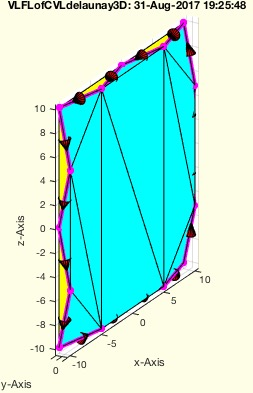% VLFLofCVLdelaunay3D(CVL) - returns the top and below tesselation for ONE closed vertex list which cuts a convex solid into two parts% (by Tim Lueth, VLFL-Lib, 2017-AUG-28 as class: SURFACES)% % In contrast to VLFLofVLELdelaunay3D, this fnctn VLFLofCVLdelaunay3D is % limited to the vertex points that are used in CVL. Based on the VL a % convex solid is created and separted into two halfs/parts by the given % EL. This works currently in 3D only for one closed contour. (Status of: % 2017-08-31)% % Introduced first in SolidGeometry 4.1% % See also: VLedgeNormal, VLFLofCVLdelaunay2D, VLFLofVLELdelaunay3D% % [VL,FLA,FLB]=VLFLofCVLdelaunay3D([CVL])% === INPUT PARAMETERS ===% CVL: Closed Polygon Vertex List% === OUTPUT RESULTS ======% VL: Vertex List% FLA: Facet List Above/Front of EL (CYAN)% FLB: Facet List Below/Back of EL (YELLOW)% % EXAMPLE: % VLFLofCVLdelaunay3D(VLsample(12))% % See also: VLedgeNormal, VLFLofCVLdelaunay2D, VLFLofVLELdelaunay3D% % % Copyright 2017 Tim C. Lueth
###### CVLofVLEL(VL,EL)- returns a closed polygon vertex list from a vertex list and an edge list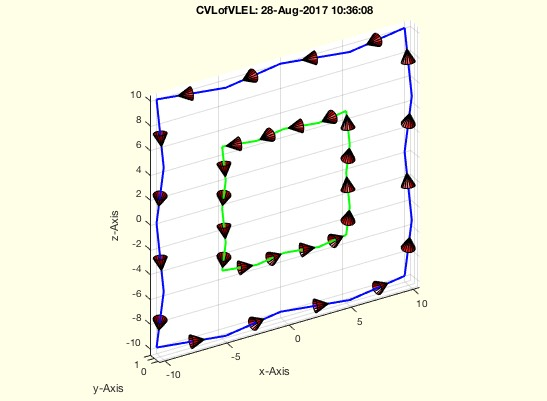% CVLofVLEL(VL,EL) - returns a closed polygon vertex list from a vertex list and an edge list% (by Tim Lueth, VLFL-Lib, 2017-AUG-28 as class: CLOSED POLYGON LISTS)% % same as CPLofPLEL (Status of: 2017-08-28)% % Introduced first in SolidGeometry 4.1% % See also: CPLofPLEL, VLELofCVL, CILofEL, CVLofVLCIL% % CVL=CVLofVLEL(VL,EL)% === INPUT PARAMETERS ===% VL: Vertex List% EL: Edge list% === OUTPUT RESULTS ======% CVL: closed polygon vertex list% % EXAMPLE: % [VL,EL]=VLELofCVL(VLsample(18)); CVLofVLEL(VL,EL)% % See also: CPLofPLEL, VLELofCVL, CILofEL, CVLofVLCIL% % % Copyright 2017 Tim C. Lueth
###### VLFLofVLELdelaunay3D(OVL,EL)- returns the top and below tesselation for ONE closed vertex list which cuts a convex solid into two parts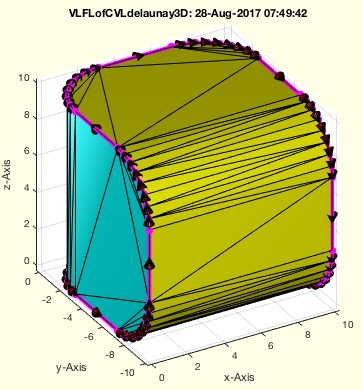% VLFLofVLELdelaunay3D(OVL,EL) - returns the top and below tesselation for ONE closed vertex list which cuts a convex solid into two parts% (by Tim Lueth, VLFL-Lib, 2017-AUG-28 as class: SURFACES)% % In contrast to VLFLofCVLdelaunay3D, this fnctn VLFLofVLELdelaunay3D is % not limited to the vertex points that are used in CVL. Based on the VL % a convex solid is created and separted into two halfs/parts by the % given EL. This works currently in 3D only for one closed contour. % (Status of: 2017-08-30)% % Introduced first in SolidGeometry 4.1% % See also: VLedgeNormal, VLFLofCVLdelaunay2D, VLFLofCVLdelaunay3D% % [VL,FLA,FLB]=VLFLofVLELdelaunay3D([OVL,EL])% === INPUT PARAMETERS ===% OVL: Original Vertex List% EL: Edge List% === PROPERTY NAMES =====% 'nowarn' : if used; the planar warning is off% === OUTPUT RESULTS ======% VL: Vertex List% FLA: Facet List Above/Front of EL (CYAN)% FLB: Facet List Below/Back of EL (YELLOW)% % EXAMPLE: % A=SGsample(5); EL=ELsort(FEofSG(A)); VLFLofVLELdelaunay3D(A.VL,EL);% A=SGsample(19); EL=ELsort(FEofSG(A)); VLFLofVLELdelaunay3D(A.VL,EL);% AVL=VLofCVL(VLsample(17)); AEL=ELofCVL(AVL); % VLFLofVLELdelaunay3D(AVL,AEL)% AVL=VLofCVL(VLsample(15)); AEL=ELofCVL(AVL); % VLFLofVLELdelaunay3D(AVL,AEL)% AVL=VLofCVL(VLsample(12)); AEL=ELofCVL(AVL); % VLFLofVLELdelaunay3D(AVL,AEL)% % See also: VLedgeNormal, VLFLofCVLdelaunay2D, VLFLofCVLdelaunay3D% % % Copyright 2017 Tim C. Lueth
###### CVLplots(CVL,c,w,t,b,f)- similar to CVLplot but shows optionally the direction of the line segments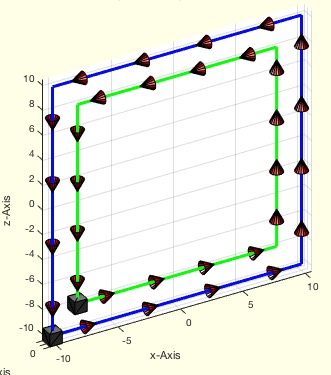% CVLplots(CVL,c,w,t,b,f) - similar to CVLplot but shows optionally the direction of the line segments% (by Tim Lueth, VLFL-Lib, 2017-AUG-28 as class: VISUALIZATION)% % This procedure is different to VLELplot (Status of: 2017-08-28)% % Introduced first in SolidGeometry 4.1% % See also: VLELplots, CPLplot, PLplot, VLplot, CVLplot% % h=CVLplots(CVL,[c,w,t,b,f])% === INPUT PARAMETERS ===% CVL: Several closed vertex list separated by nan% c: Optional color of desired edges; default changing colors% w: Optional line width; default is 1% t: Size of a cone shaped arrow tip, default is ''=invisible% b: Size of a cube showing the start point; default is ''=invisible% f: Size of a ring showing the end point; default is ''=invisible% === OUTPUT RESULTS ======% h: handle to drawing elements% % EXAMPLE: % SGfigure; view(-30,30); CVLplots(VLsample(18),'',3,1);% SGfigure; view(-30,30); CVLplots(VLsample(18),'',3,1,1);% SGfigure; view(-30,30); CVLplots(VLsample(18),'',3,1,1,1);% % See also: VLELplots, CPLplot, PLplot, VLplot, CVLplot% % % Copyright 2017 Tim C. Lueth
###### VLELofCVL(CVL)- converts nan separated succeeding vertex lists into a vertex list and an edge list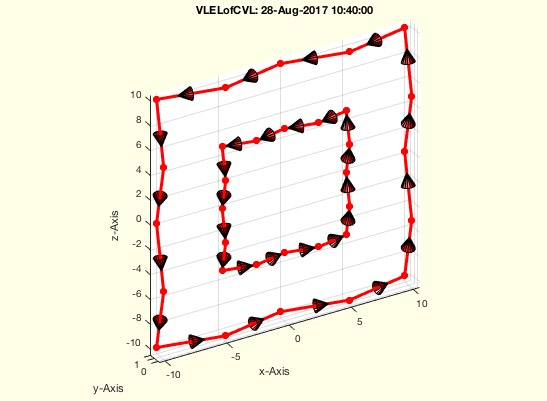% VLELofCVL(CVL) - converts nan separated succeeding vertex lists into a vertex list and an edge list% (by Tim Lueth, VLFL-Lib, 2017-AUG-27 as class: AUXILIARY PROCEDURES)% % This fnctn removes the NaN from the original point list. The % orientation (cw/ccw) is not changed. It is exactly the same as % PLELofCPL (Status of: 2017-08-28)% % Introduced first in SolidGeometry 4.1% % See also: ELofCVL, PLELofCPL, CVLofVLEL% % [VL,EL]=VLELofCVL(CVL)% === INPUT PARAMETERS ===% CVL: NAN Separated Vertex List% === OUTPUT RESULTS ======% VL: Vertex List (without NaN)% EL: Edge list% % EXAMPLE: % [VL,EL]=VLELofCVL(VLsample(19)); CVL=CVLofVLEL(VL,EL); % VLELofCVL(VLsample(19));% % See also: ELofCVL, PLELofCPL, CVLofVLEL% % % Copyright 2017 Tim C. Lueth
###### VLof2VLnonmanifold(VLA,VLB)- returns vertex list and indices of non manifold vertices on TWO DIFFERENT vertex lists% VLof2VLnonmanifold(VLA,VLB) - returns vertex list and indices of non manifold vertices on TWO DIFFERENT vertex lists% (by Tim Lueth, VLFL-Lib, 2017-AUG-25 as class: SURFACES)% % This fnctn is able to detect potential problems in SGbool before % execution (Status of: 2017-08-25)% % Introduced first in SolidGeometry 4.1% % See also: VLmindxyz, VLofcorruptedFL% % [NML,nma,nmb]=VLof2VLnonmanifold(VLA,VLB)% === INPUT PARAMETERS ===% VLA: Vertex list A% VLB: Vertex list B% === OUTPUT RESULTS ======% NML: Non manifold vertices; identical points used in both solids% nma: % nmb: % % EXAMPLE: % [A,B]=CSGsample(12); % VLof2VLnonmanifold(roundn(A.VL,1e-4),roundn(B.VL,1e-4))% % See also: VLmindxyz, VLofcorruptedFL% % % Copyright 2017 Tim C. Lueth
###### FLofELinFL(FL,EL,)- finds one ore more edges in a facets list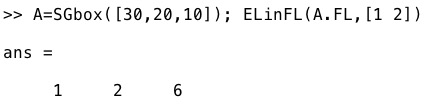% FLofELinFL(FL,EL,) - finds one ore more edges in a facets list% (by Tim Lueth, VLFL-Lib, 2017-AUG-24 as class: AUXILIARY PROCEDURES)% % this is a search fnctn ismember(FL,EL) (Status of: 2017-08-25)% % Introduced first in SolidGeometry 4.1% % See also: FLunique, ELinFL, ELofFL, ELofFLborder% % [FL,ci]=FLofELinFL(FL,EL,[])% === INPUT PARAMETERS ===% FL: Facet list% EL: Edge List% === OUTPUT RESULTS ======% FL: Faces that contain the edges% ci: selection index% % EXAMPLE: % A=SGbox([30,20,10]); ELinFL(A.FL,[1 2])% % See also: FLunique, ELinFL, ELofFL, ELofFLborder% % % Copyright 2017 Tim C. Lueth
###### VLmindxyz(VL,Name,FL,grid)- returns the minimal distance larger than zero% VLmindxyz(VL,Name,FL,grid) - returns the minimal distance larger than zero % (by Tim Lueth, VLFL-Lib, 2017-AUG-24 as class: ANALYTICAL GEOMETRY)% % The smallest number in matlab different from zero is eps ~1e-15;% The typical arithmetic error of ordinary calculation is ~1e-12;% The smallest trigonometric number bigger than zero is eps2 ~1e-07;% A boolean surface operation should use coordinate grids ~1e-03;% The crossing points NPL of those coordinate would be up to ~1e-12;% Rounding limits > 1e-12 will create new point in triangulation; % (Status of: 2017-08-24)% % Introduced first in SolidGeometry 4.1% % See also: VLmindist, eps, eps2, epsdyn, SGboolanalyze% % [dmin,dmm]=VLmindxyz(VL,[Name,FL,grid])% === INPUT PARAMETERS ===% VL: vector list% Name: Optional Name string for warnings and verbose print% FL: Optional Facet list to get information on Area and collapsed facets% grid: Optional Grid size to estimate the problems by grid rounding% === OUTPUT RESULTS ======% dmin: absolute minimal distance% dmm: abolute maximum dimension% % EXAMPLE: % VLmindxyz(SGsample(5)), % Shows the minimal point distance for a half % sphere% % See also: VLmindist, eps, eps2, epsdyn, SGboolanalyze% % % Copyright 2017 Tim C. Lueth
###### SGdelaunay(A,alpha)- returns a solid with delaunay surfaces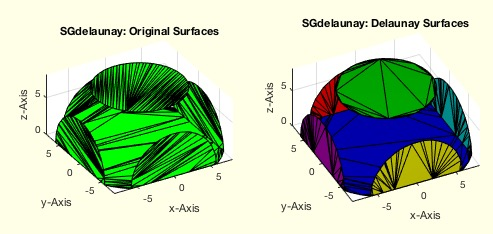% SGdelaunay(A,alpha) - returns a solid with delaunay surfaces% (by Tim Lueth, VLFL-Lib, 2017-AUG-24 as class: SURFACES)% % This fnctn takes all planar surfaces and creates a new delaunay % tesselation for this planar surfaces% ATTENTION. The new facet list will not change in size!% ATTENTION. Sometimes the new surface is only mirrored on turned% ATTENTION. The alpha value will smoothen the surface but can lead to % undesired side effects% A larger alpha value will flatten spherical surfaces and create % undesired spherical shortcuts! (Status of: 2017-08-24)% % Introduced first in SolidGeometry 4.1% % See also: SGreduceVLFL, SGretesselate% % SGN=SGdelaunay(A,[alpha])% === INPUT PARAMETERS ===% A: Original Solid Geometry% alpha: feature surface angle; default is 0.01% === OUTPUT RESULTS ======% SGN: Solid Geometry with delaunay surfaces% % EXAMPLE: % A=SGsample(5); B=SGcutBB(A,BBofSG(A)*0.8,0.1,'bool');% SGdelaunay(B,0.01)% % See also: SGreduceVLFL, SGretesselate% % % Copyright 2017 Tim C. Lueth
###### SGtransrelT(SG,T0,T)- tranforms a solid relative to known one% SGtransrelT(SG,T0,T) - tranforms a solid relative to known one% (by Tim Lueth, VLFL-Lib, 2017-AUG-22 as class: SURFACES)% % % SGN=SGtransT(SG,T0*T*(eye(4)/T0));% (Status of: 2017-08-23)% % Introduced first in SolidGeometry 4.1% % See also: SGtrans, SGtrans0, SGtrans1, SGtransP, SGtransR, % SGtransrelSG, SGtransT% % SGN=SGtransrelT(SG,T0,T)% === INPUT PARAMETERS ===% SG: Solid Geometry% T0: Reference Frame Matrix or string for SGTget% T: Relative Transformation Frame% === OUTPUT RESULTS ======% SGN: Spatial transformed solid% % See also: SGtrans, SGtrans0, SGtrans1, SGtransP, SGtransR, % SGtransrelSG, SGtransT% % % Copyright 2017 Tim C. Lueth
###### CVLclipT(CVL,T,ax)- returns a clipped CVL relative to a HT matrix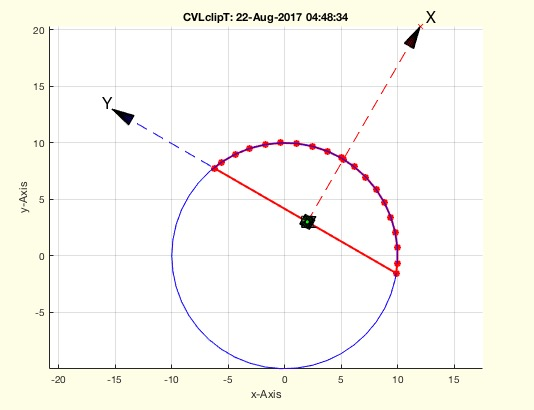% CVLclipT(CVL,T,ax) - returns a clipped CVL relative to a HT matrix% (by Tim Lueth, VLFL-Lib, 2017-AUG-22 as class: ANALYTICAL GEOMETRY)% % Be careful if more than one axis is clipped! unexpected results ..... % (Status of: 2017-08-22)% % Introduced first in SolidGeometry 4.1% % See also: CVLinsertT% % CVLC=CVLclipT(CVL,T,ax)% === INPUT PARAMETERS ===% CVL: Closed Polyggon in 3D% T: Frame matrix% ax: clipping area; [1 0 0]; [-1 0 0]; [0 1 0]; [0 -1 0]% === OUTPUT RESULTS ======% CVLC: Clipped contour% % EXAMPLE: % CVLclipT(VLaddz(PLcircle(10)),TofR(rot(0,0,pi/3),[2 3 0]),[0 0 0])% CVLclipT(VLaddz(PLcircle(10)),TofR(rot(0,0,pi/3),[2 3 0]),[-1 0 0])% CVLclipT(VLaddz(PLcircle(10)),TofR(rot(0,0,pi/3),[2 3 0]),[0 1 0])% % See also: CVLinsertT% % % Copyright 2017 Tim C. Lueth
###### CVLinsertT(CVL,T)- inserts the x/y crossing points of a CVL and a HT matrix of a plane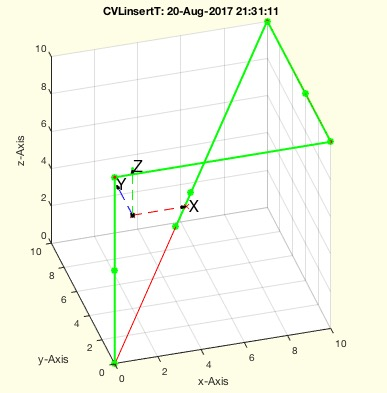% CVLinsertT(CVL,T) - inserts the x/y crossing points of a CVL and a HT matrix of a plane% (by Tim Lueth, VLFL-Lib, 2017-AUG-20 as class: ANALYTICAL GEOMETRY)% % In case that a CVL should be cut by T matrix planes into two contour, % it is helpful to create and insert 3D splitpoint into the original CVL % (Status of: 2017-08-20)% % Introduced first in SolidGeometry 4.1% % See also: CVLremstraightAmin% % NCVL=CVLinsertT(CVL,T)% === INPUT PARAMETERS ===% CVL: CVL% T: T matrix% === OUTPUT RESULTS ======% NCVL: NCVL matrix with crosing points with the xy plane% % EXAMPLE: % CVLinsertT(VLsample(8),TofP([2 4 5]))% % See also: CVLremstraightAmin% % % Copyright 2017 Tim C. Lueth
###### exp_2017_08_21 (SG)- EXPERIMENT THAT IS IN FACT SGofCVLslices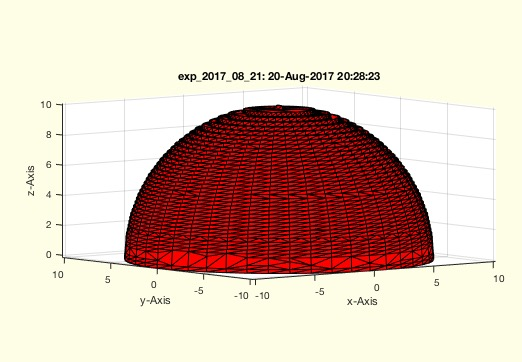% exp_2017_08_21 (SG) - EXPERIMENT THAT IS IN FACT SGofCVLslices% (by Tim Lueth, VLFL-Lib, 2017-AUG-20 as class: EXPERIMENTS)% % Introduced first in SolidGeometry 4.1% % See also: SGofCVLslices% % exp_2017_08_21([SG])% === INPUT PARAMETERS ===% SG: % % See also: SGofCVLslices% % % Copyright 2017 Tim C. Lueth
###### SGseparatebyT(SG,T)- separates a solid into surfaces that are clearly below or above of a xy-plane% SGseparatebyT(SG,T) - separates a solid into surfaces that are clearly below or above of a xy-plane% (by Tim Lueth, VLFL-Lib, 2017-AUG-20 as class: SURFACES)% % In contrast to SGcutT, when two solids are separated by a plane, this % fnctn SGseparatebyT raytraces the ez vector of T to identify solids % that are infront or behind the T coordinate system. It separates the % surfaces into "below", "above" or "undefined".% The solids of SGcutT would be considered as separated solids too. % (Status of: 2017-08-25)% % Introduced first in SolidGeometry 4.1% % See also: SGsurfaces, SGcutT% % [SGX,SGA,SGB,SGC]=SGseparatebyT(SG,T)% === INPUT PARAMETERS ===% SG: Solid Geoemtry% T: Separating frame/ plane% === OUTPUT RESULTS ======% SGX: Surface that enclosed T (magenta); uncut% SGA: Surfaces clearly ABOVE T (red)% SGB: Surfaces clearly BELOW T (green)% SGC: Surfaces that below and above of T (white)% % EXAMPLE: % A=SGsample(5); T=TofP([0 0 3]); B=SGbladeofCVL(CVLofSGT(A,T),2,2); % A=SGboolTL(A,'-',B); SGseparatebyT(A,T)% % A=SGsample(5); T=TofP([0 0 3]); [A,B]=SGcutT(A,T); SGfigure; % SGplot(A,'r'); SGplot(B,'g'); % SGseparatebyT({A,B},T)% % SGseparatebyT(SGsample(5),T)% % See also: SGsurfaces, SGcutT% % % Copyright 2017 Tim C. Lueth
###### VLFLofCVLdelaunay2D(CVL);- returns a triangulation for an almost planar CVL% VLFLofCVLdelaunay2D(CVL); - returns a triangulation for an almost planar CVL% (by Tim Lueth, VLFL-Lib, 2017-AUG-20 as class: SURFACES)% % This fnctn works only for planar CVL (possible in different planes) and % if CVL contains only open contours and there is no curvature larger % than 90 degree. (Status of: 2017-08-28)% % Introduced first in SolidGeometry 4.1% % See also: PLFLofCPLdelaunay% % [VL,FL]=VLFLofCVLdelaunay2D(CVL);% === INPUT PARAMETERS ===% CVL): 3D closed contour vertex list% === OUTPUT RESULTS ======% VL: Vertex list% FL: Facet List% % EXAMPLE: % VLFLofCVLdelaunay(VLsample(13)+5);% VLFLofCVLdelaunay(VLsample(19)+5);% % % See also: PLFLofCPLdelaunay% % % Copyright 2017 Tim C. Lueth
###### SGbladeofCVL(CVL,sl,ad)- returns a solid for an almost planar CVL the closing facets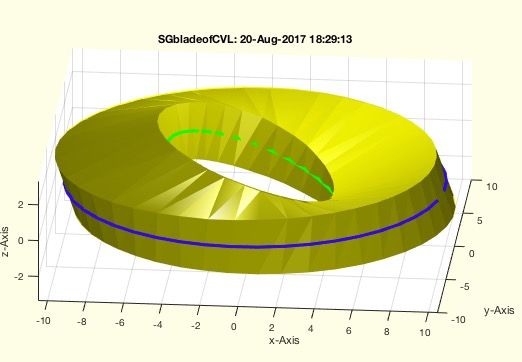% SGbladeofCVL(CVL,sl,ad) - returns a solid for an almost planar CVL the closing facets% (by Tim Lueth, VLFL-Lib, 2017-AUG-20 as class: SURFACES)% % Introduced first in SolidGeometry 4.1% % See also: VLFLofCVLdelaunay2D, SGofSurface% % SG=SGbladeofCVL(CVL,[sl,ad])% === INPUT PARAMETERS ===% CVL: Closed vertex list; open polygons% sl: Blade thickness; default=0.3% ad: size growing; default=0;% === OUTPUT RESULTS ======% SG: Solid Geometry of a blade% % EXAMPLE: % SGbladeofCVL(VLsample(19),3)% SGbladeofCVL([VLaddz(PLcircle(10));nan nan nan; % VLtransR(VLaddz(PLcircle(5)),rot(0,pi/8,0))],3)% % See also: VLFLofCVLdelaunay2D, SGofSurface% % % Copyright 2017 Tim C. Lueth
###### TofT(T0,R,P)- returns a T matrix defined relative to another T matrix% TofT(T0,R,P) - returns a T matrix defined relative to another T matrix% (by Tim Lueth, VLFL-Lib, 2017-AUG-20 as class: ANALYTICAL GEOMETRY)% % This fnctn help to specify a new matrix relative to an existing one. % This is helpful to define cutting planes for SGcutT for example.% In fact it is the same as T1=T0*TofR(R,P) but may be easier to % understand. (Status of: 2017-08-20)% % Introduced first in SolidGeometry 4.1% % See also: TofR, TofVL, TPL, TofDPhiH, T3ofT2, T3P, T2P, TofP, TofPez, % TofPEul% % T1=TofT(T0,[R,P])% === INPUT PARAMETERS ===% T0: Original Matrix% R: Rotation matrix relative to the T axis; scalar=phiz% P: Translation vector relative to the T axis; scalar=z% === OUTPUT RESULTS ======% T1: resulting new T Matrix% % EXAMPLE: % TofT(TofP([10 10 10]),10)% TofT(TofP([10 10 10]),rot(0,0,pi))% TofT(TofP([10 10 10]),pi/2,2)% % See also: TofR, TofVL, TPL, TofDPhiH, T3ofT2, T3P, T2P, TofP, TofPez, % TofPEul% % % Copyright 2017 Tim C. Lueth
###### CVLremstraightAmin(CVL,Amin)- removes points with a minal distance/area to a predecessor or successor% CVLremstraightAmin(CVL,Amin) - removes points with a minal distance/area to a predecessor or successor% (by Tim Lueth, VLFL-Lib, 2017-AUG-20 as class: CLOSED POLYGON LISTS)% % This fnctn seperates individual CPLs by nan and processes them using % VLremstraightAmin The result is always an open end CPL! Ohter fnctn % such as CVLinsertT or CPLaddauxpoints insert additional points into a % contour (Status of: 2017-08-22)% % Introduced first in SolidGeometry 4.1% % See also: VLremstraightAmin, CVLremstraight, CVLinsertT% % NVL=CVLremstraightAmin(CVL,[Amin])% === INPUT PARAMETERS ===% CVL: Closed Polygon Line in 2D or 3D% Amin: Minimal Area between two succeeding points; default is 1e-3;% === OUTPUT RESULTS ======% NVL: New Vertex List% % EXAMPLE: % CVLremstraightAmin(VLaddz([PLcircle(10,100); nan % nan;PLcircle(1,100)]),1)% CVLremstraightAmin([PLcircle(10,100); nan nan;PLcircle(1,100)],1)% % See also: VLremstraightAmin, CVLremstraight, CVLinsertT% % % Copyright 2017 Tim C. Lueth
###### VLremstraightAmin(CVL,Amin)- removes points with a minal distance/area to a predecessor or successor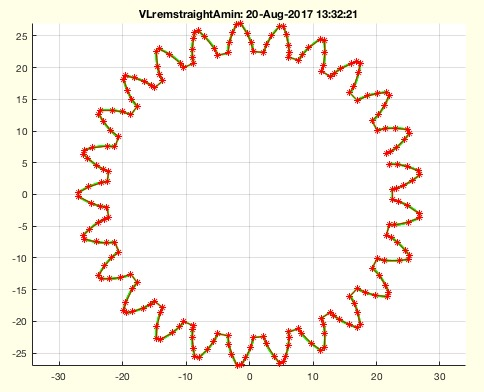% VLremstraightAmin(CVL,Amin) - removes points with a minal distance/area to a predecessor or successor% (by Tim Lueth, VLFL-Lib, 2017-AUG-19 as class: CLOSED POLYGON LISTS)% % VLremstraightCVL is an impressive simple but efficient method. % Nevertheless it fails if the point density is too tight. This fnctn % VLremstraightAmin adds the removal of each second (!) point that is too % tight.% The result is always open at the end! (Status of: 2017-08-20)% % Introduced first in SolidGeometry 4.1% % See also: CVLremstraightAmin% % [NVL,ci]=VLremstraightAmin(CVL,[Amin])% === INPUT PARAMETERS ===% CVL: Closed Polygon Line in 2D or 3D% Amin: Minimal Area between two succeeding points; default is 1e-3;% === OUTPUT RESULTS ======% NVL: New Vertex List% ci: mapping index from original to new% % EXAMPLE: % VL=VLaddz(PLcircle(1,10000)); VLremstraightAmin(roundn(VL,1e-7),1e-3); % VLremstraightAmin(VLaddz(PLsample(3)),1e-1);% VLremstraightAmin(VLaddz(CPLsample(19)),1e-1);% VLremstraightAmin(VLaddz(CPLsample(19)),1e-2% VLremstraightAmin(VLaddz(VLsample(15)),1e-1);% VLremstraightAmin(VLaddz(CPLsample(21)),1e-1);% % See also: CVLremstraightAmin% % % Copyright 2017 Tim C. Lueth
###### exp_2017_08_18- EXPERIMENT to show numerical resolution effects% exp_2017_08_18 - EXPERIMENT to show numerical resolution effects% (by Tim Lueth, VLFL-Lib, 2017-AUG-19 as class: EXPERIMENTS)% % Introduced first in SolidGeometry 4.1% % See also: VLremstraightAmin, CVLremstraightAmin% % exp_2017_08_18% % See also: VLremstraightAmin, CVLremstraightAmin% % % Copyright 2017 Tim C. Lueth
###### epsofVL(NVL,n)- returns the minimal points distance in a set of vertices or two groups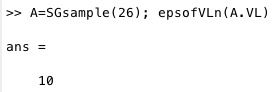% epsofVL(NVL,n) - returns the minimal points distance in a set of vertices or two groups% (by Tim Lueth, VLFL-Lib, 2017-AUG-17 as class: AUXILIARY PROCEDURES)% % The fnctn can also be called using two VL or two SG. The fnctn has % quadratic effort. It wil become very slow for large vertex lists. % (Status of: 2017-08-17)% % Introduced first in SolidGeometry 4.1% % See also: eps, eps2% % [e,ea,eb]=epsofVL(NVL,[n])% === INPUT PARAMETERS ===% NVL: Vertex list with appended split points% n: number to split the vertex list into 2 parts [1:n,:] & [n+1:end,:]% === OUTPUT RESULTS ======% e: smallest number larger 0 between the points% ea: epsilon within the first group% eb: epsilon within the second group% % EXAMPLE: % A=SGsample(5); SGfigure(A); view(-30,30); epsofVL(A.VL)% A=SGsample(26); SGfigure(A); view(-30,30); epsofVL(A.VL)% A=SGsample(25); SGfigure(A); view(-30,30); epsofVL(A.VL)% A=SGsample(5); SGfigure(A); view(-30,30); [a,b,c]=epsofVL(A.VL,500)% A=SGsample(26); SGfigure(A); view(-30,30); [a,b,c]=epsofVL(A.VL,5)% % See also: eps, eps2% % % Copyright 2017 Tim C. Lueth
###### FLunique(FL)- returns a FL without copies of circshifted rows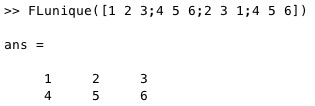% FLunique(FL) - returns a FL without copies of circshifted rows% (by Tim Lueth, VLFL-Lib, 2017-AUG-17 as class: SURFACES)% % Introduced first in SolidGeometry 4.1% % See also: ELunique, ELuniqueofFL% % FL=FLunique(FL)% === INPUT PARAMETERS ===% FL: Facet list% === OUTPUT RESULTS ======% FL: Facet list with unique rows;% % EXAMPLE: % FLunique([1 2 3;4 5 6;2 3 1;4 5 6])% % See also: ELunique, ELuniqueofFL% % % Copyright 2017 Tim C. Lueth
###### VLmindist(VL,epsr)- returns the minimal distance larger than zero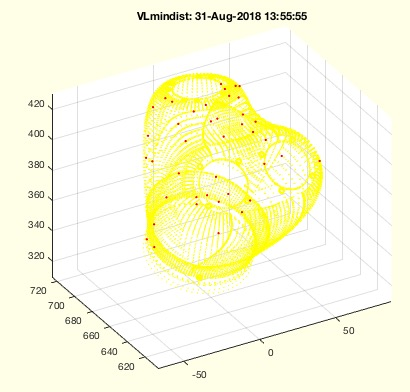% VLmindist(VL,epsr) - returns the minimal distance larger than zero % (by Tim Lueth, VLFL-Lib, 2017-AUG-17 as class: ANALYTICAL GEOMETRY)% % After reading in a STL file, there is a need to check the minmal % distances between the vertices.% If the minimal distance is smaller than the minimal value of Matlab, % eps, accuracy errors will occur. (Status of: 2018-08-31)% % Introduced first in SolidGeometry 4.1% % See also: eps, eps2, epsdyn, VLmindxyz, SGshortopti, % VLcheckvertexaccuracy% % [dmm,dmin]=VLmindist(VL,[epsr])% === INPUT PARAMETERS ===% VL: vector list% epsr: optional rounding before; default is 0, i.e. no rounding% === OUTPUT RESULTS ======% dmm: absolute minimal distance% dmin: minmal distance per column% % EXAMPLE: % VLmindist(rand(1000000,1)) % linear minimal random distance% VLmindist(rand(1000000,2)) % quadratic minimal random distance% VLmindist(rand(1000000,3)) % cubic minimal random distance% % loadweb JACO_robot.mat% VLmindist(JC61.VL)% % See also: eps, eps2, epsdyn, VLmindxyz, SGshortopti, % VLcheckvertexaccuracy% % % Copyright 2017-2018 Tim C. Lueth
###### SGplotparts(A,c,Fs,si)- plots the overlapping parts of a solid separately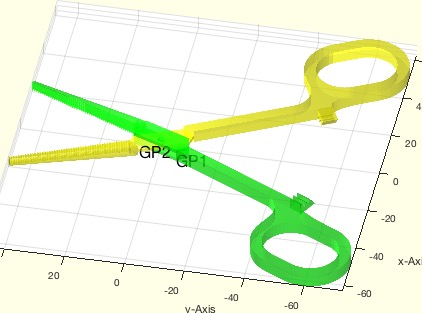% SGplotparts(A,c,Fs,si) - plots the overlapping parts of a solid separately% (by Tim Lueth, VLFL-Lib, 2017-AUG-16 as class: SURFACES)% % The result of SGanalyzeGroupParts is a struct with a field SG. It % contains overlapping objects that form a melted/joined solid and could % be group melted (Status of: 2017-08-16)% % Introduced first in SolidGeometry 4.1% % See also: SGanalyzeGroupParts, SGplot, SGplotsurfaces% % h=SGplotparts(A,[c,Fs,si])% === INPUT PARAMETERS ===% A: Solid or Group Part Solid (A.SG)% c: color or colorstring such as 'rbwcr'% Fs: Font size for Descriptor; default is 16% si: selector for group parts% === OUTPUT RESULTS ======% h: handle to graphic objects% % EXAMPLE: close all; SGfigure; view(30,30); SGplotparts(SGsample(17));% GP=SGanalyzeGroupParts(SGsample(17));% close all; SGfigure; view(30,30); SGplotparts(GP);% close all; SGfigure; view(30,30); SGplotparts(GP,'wr');% close all; SGfigure; view(30,30); SGplotparts(GP,'wr',20);% close all; SGfigure; view(30,30); SGplotparts(GP,'wr',20,1);% close all; SGfigure; view(30,30); SGplotparts(GP,'wr',20,2);% % See also: SGanalyzeGroupParts, SGplot, SGplotsurfaces% % % Copyright 2017 Tim C. Lueth
###### SGplotsurfaces(SG,col,Fs,si)- plots closed surface of the solids seperatly in different colors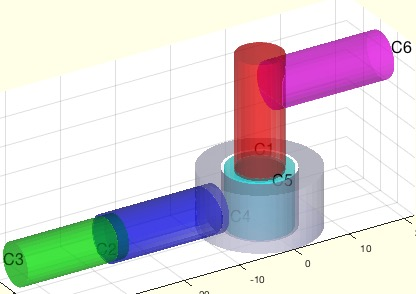% SGplotsurfaces(SG,col,Fs,si) - plots closed surface of the solids seperatly in different colors% (by Tim Lueth, VLFL-Lib, 2017-AUG-16 as class: USER INTERFACE)% % very similar to SGsurfaceplot, but more a clear plotting fnctn% (Status of: 2017-08-16)% % Introduced first in SolidGeometry 4.1% % See also: SGseparate% % h=SGplotsurfaces(SG,[col,Fs,si])% === INPUT PARAMETERS ===% SG: Solid Geoemtry% col: color force; default is '';% Fs: Font size for descriptors; default is 0% si: surface selectors% === OUTPUT RESULTS ======% h: handle to drawing% % See also: SGseparate% % % Copyright 2017 Tim C. Lueth
###### SGchamfer(SG,ph,ed,r,stype,posit,shape,hole)- chamfers the edges of a 2.5D Solid Geometry% SGchamfer(SG,ph,ed,r,stype,posit,shape,hole) - chamfers the edges of a 2.5D Solid Geometry% (by Tim Lueth, VLFL-Lib, 2017-AUG-16 as class: SURFACES)% % Optimized in Dakhla, Marocco. It does not returns as perfect edges as % SGofCPLzchamfer but is the same as SGchamfer! Used first with negative % values by Samuel Detzel!% if r>0; r must be larger than abs(ph)*1.5% ATTENTION: If r is reduced automatically by tangentcirc, ph can be only % r/1.5, or r must be zero! (Status of: 2018-04-16)% % Introduced first in SolidGeometry 4.1% % See also: PLchamfer, SGofCPLzchamfer, SGof2CPLzheurist, CPLradialEdges% % SGN=SGchamfer(SG,[ph,ed,r,stype,posit,shape,hole])% === INPUT PARAMETERS ===% SG: Solid Geometry with just two different z values% ph: edge/phase size default is 0.3; [r z], [r zs ze] is also valid% ed: curved edges; default is true% r: radius for radial edges; default is ph*1.5 % stype: default is 'angle'; see SGof2CPLzheurist% posit: 'both', 'start', 'end' or calculated from ph% shape: PL or 'line' (default), 'circ', see PLchamfer for all values% hole: "norm", "outw", "hole", see CPLgrow for all values% === OUTPUT RESULTS ======% SGN: % % EXAMPLE: % SGchamfer(SGsample(2))% SGchamfer(SGofCPLz(PLcircleoval(10,'',20,5),10))% SGchamfer(SGofCPLz(PLstar(10),10),0.3,'')% SGchamfer(SGofCPLz(PLstar(10),10),0.3,'',2)% SGchamfer(SGofCPLz(PLstar(10),10),0.3,'',-0.5)% SGchamfer(SGofCPLz(PLstar(10),10),-1)% SGchamfer(SGlinkage(5,30,10),0.3)% SGchamfer(SGsample(10),-2,false,0)% SGchamfer(SGsample(10),-2,true,0)% SGchamfer(SGsample(10),-2,true,0,'center')% % See also: PLchamfer, SGofCPLzchamfer, SGof2CPLzheurist, CPLradialEdges% % % Copyright 2017-2018 Tim C. Lueth
###### SGbladeofPL(sl,XZL,YZL,T,XSG)- returns a solid with a shape of a cutting blade in z direction% SGbladeofPL(sl,XZL,YZL,T,XSG) - returns a solid with a shape of a cutting blade in z direction% (by Tim Lueth, VLFL-Lib, 2017-AUG-16 as class: SURFACES)% % This fnctn create a big variety of blade structures depending on the % input values;% (Status of: 2017-08-16)% % Introduced first in SolidGeometry 4.1% % See also: SGbool, SGinsertCut, SGcutBB, SGinsertPeghole% % SG=SGbladeofPL([sl,XZL,YZL,T,XSG])% === INPUT PARAMETERS ===% sl: thickness of the blade% XZL: z or [x z] or PL with xz values;% YZL: depth in Y% T: Transformation Matrix% XSG: Final Crossing Solid to limit the outer shape% === OUTPUT RESULTS ======% SG: Cutting Blade Slid Geometry% % EXAMPLE: % SGbladeofPL(3,10)% SGbladeofPL(0.5,10)% SGbladeofPL(1,[10,10])% SGbladeofPL(0.05,PLcircle(10))% SGbladeofPL(0.05,CPLofPL(PLcircle(10)))% A=SGbladeofPL(0.5,CPLofPL(PLcircle(3)),30,TofP([0 0 5])); % SGboolTL(SGsample(5),'-',A)% SGbladeofPL(0.05,CPLofPL(PLcircle(3,8)),30,TofP([0 0 5]),SGsample(5));% % See also: SGbool, SGinsertCut, SGcutBB, SGinsertPeghole% % % Copyright 2017 Tim C. Lueth
###### SGboolTL(A,op,B,Amin)- returns a Boolean operation including re T esse L ation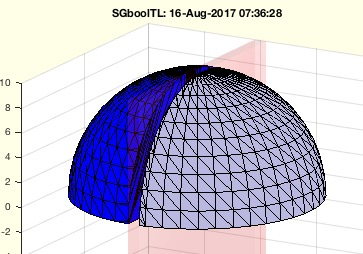% SGboolTL(A,op,B,Amin) - returns a Boolean operation including re T esse L ation% (by Tim Lueth, VLFL-Lib, 2017-AUG-16 as class: SURFACES)% % This fnctn calls SGbool but performs preprocessing and postprocessing. % The syntax is changed from (Operation, A, B) to (A, Operation, B)% 1. Both argoment solids and the resulting solids get a retesselation % using a minimal facet area of Amin% 2. Argument Solid B is grown by 1e-5 % This is done to make sure that identical points,faces, solids can be % processed.% If SGbool fails, there is always a numerical problem.% The retesselation will create sometimes faces of a minimal area just to % turn the edges of some facets. This is a principal problem until a % global retesselation solution is implemented if exists at all.% % This fnctn will be integrated into SGbool soon, since the type of the % second argument will help to distinguish between two types:% SGbool ('+',A,B) or SGbool(A,'+',B) ==> SGboolTL(A,'+',B)% % BTW: CATIA LIMITS THE SPATIAL RESOLUTION TO 0.01mm, ie. 1e-2% (Status of: 2017-08-16)% % Introduced first in SolidGeometry 4.1% % See also: SGbool, SGretesselate, SGgrow% % SG=SGboolTL(A,op,B,[Amin])% === INPUT PARAMETERS ===% A: Solid A% op: Boolean Operator% B: Solid B% Amin: Minimal Area for tessealtion; default 0.01% === OUTPUT RESULTS ======% SG: % % EXAMPLE: % A=SGsample(26); SGboolTL(A,'+',SGtransP(A,[0 0 10]))% A=SGsample(5); B=SGbox([1,20,20]); SGboolTL(A,'-',B);% A=SGsample(27); B=SGbox([1,20,20]); SGboolTL(A,'-',B);% A=SGsample(5); B=SGbox([1,15,15]); SGboolTL(A,'-',B);% A=SGsample(26); B=SGoftext('TIM',[20,4,2]); % SGboolTL(A,'-',SGtransrelSG(B,A,'center','aligntop',1));% % See also: SGbool, SGretesselate, SGgrow% % % Copyright 2017 Tim C. Lueth
###### SGinsertPeghole(SG,T,sl,sx,ad)- returns a solid with an inserted peg and hole connector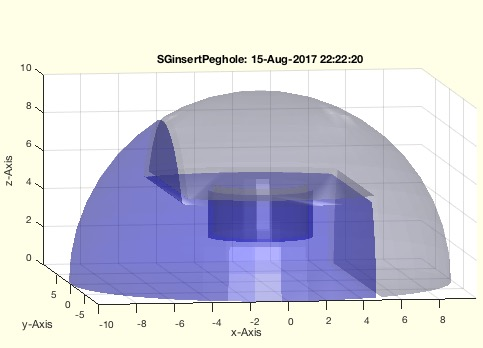% SGinsertPeghole(SG,T,sl,sx,ad) - returns a solid with an inserted peg and hole connector% (by Tim Lueth, VLFL-Lib, 2017-AUG-15 as class: SURFACES)% % After cutting an object using (Status of: 2017-08-15)% % Introduced first in SolidGeometry 4.1% % See also: SGbool, SGinsertCut, SGcutBB% % [SG,T,d]=SGinsertPeghole(SG,[T,sl,sx,ad])% === INPUT PARAMETERS ===% SG: Solid Geometry% T: Separation plane% sl: slot size peg and hole% sx: diameter of slot size; or CPL of peg% ad: doubled length of peg % === OUTPUT RESULTS ======% SG: Solid Geometry with a peg and a hole% T: % d: % % EXAMPLE: % [X,T]=SGinsertCut(SGsample(5),eye(4),0.3,10)% SGinsertPeghole(X,T,0.3,10,)% SGinsertPeghole(X,T,0.3,10,5)% SGinsertPeghole(X,T,0.3,PLcircle(3,4),5)% % See also: SGbool, SGinsertCut, SGcutBB% % % Copyright 2017 Tim C. Lueth
###### SGinsertCut(SG,T,sl,sx,ad)- returns a solid with an inserted cut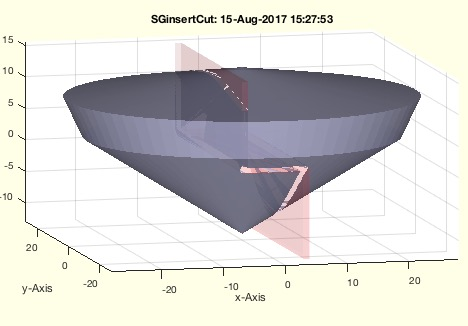% SGinsertCut(SG,T,sl,sx,ad) - returns a solid with an inserted cut% (by Tim Lueth, VLFL-Lib, 2017-AUG-15 as class: SURFACES)% % Introduced first in SolidGeometry 4.1% % See also: SGbool, SGcutBB% % [SG,T,d]=SGinsertCut(SG,[T,sl,sx,ad])% === INPUT PARAMETERS ===% SG: Solid Geometry% T: Transformation for cut; default is eye(4)% sl: cut slot size; default is 0.3% sx: transversal separation of cut; default is 0;% ad: addendum ; default is 1 mm; tbi: if negative add is added to bb of % SG% === OUTPUT RESULTS ======% SG: Solid Geoemtry, retesselated Amin=0.01% T: exact cutting point matrix% d: distance values relative to T% % EXAMPLE: % SGinsertCut(SGsample(5),'',1,4)% SGinsertCut(SGsample(5),'','',4)% SGinsertCut(SGsample(5),'','','')% SGinsertCut(SGsample(5),'','','',5)% SGinsertCut(SGsample(5),TofR(rot(0,0,pi/4)),'','',5)% % See also: SGbool, SGcutBB% % % Copyright 2017 Tim C. Lueth
###### VLFLfreeboundary(VL,FL)- returns the freeboundary of a solid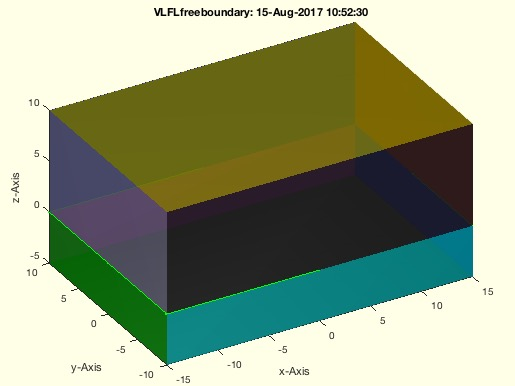% VLFLfreeboundary(VL,FL) - returns the freeboundary of a solid% (by Tim Lueth, VLFL-Lib, 2017-AUG-15 as class: SURFACES)% % Same as fnctn freeBoundary. There is a difference to ELboundaryFL or % ELofFLborder, since a triangualtion is created first that detects % doubled vertices. (Status of: 2017-08-15)% % Introduced first in SolidGeometry 4.1% % See also: VLFLplotfreeboundary, ELboundaryFL, ELofFLborder% % EL=VLFLfreeboundary(VL,FL)% === INPUT PARAMETERS ===% VL: Vertex list or Solid Geoemtry% FL: Facet List or empty% === OUTPUT RESULTS ======% EL: Edge list% % See also: VLFLplotfreeboundary, ELboundaryFL, ELofFLborder% % % Copyright 2017 Tim C. Lueth
###### PLgrowline(PL,s,edge);- converts a single open polygon into a grown contour% PLgrowline(PL,s,edge); - converts a single open polygon into a grown contour% (by Tim Lueth, VLFL-Lib, 2017-AUG-14 as class: CLOSED POLYGON LISTS)% % Introduced first in SolidGeometry 4.1% % See also: PLgrow, CPLgrow% % CPL=PLgrowline(PL,s,[edge]);% === INPUT PARAMETERS ===% PL: Open point list line% s: thickness% edge: true= rounded edges; false = simple;% === OUTPUT RESULTS ======% CPL: Closed Polygon line% % EXAMPLE: % PL=[-5 10; -5 0; +5 0; +5 -10]% PLgrowline(PL,1,true)% PLgrowline(PL,1,false)% % See also: PLgrow, CPLgrow% % % Copyright 2017 Tim C. Lueth
###### VLFLfaceAngles(VL,FL,thr)- returns the three angles for the facets of a solid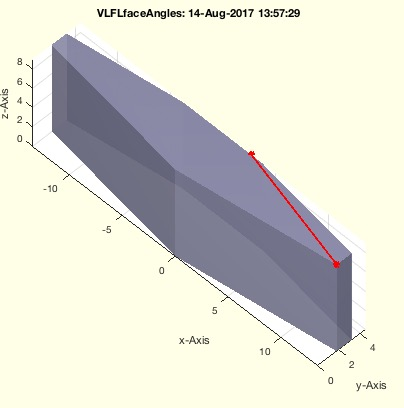% VLFLfaceAngles(VL,FL,thr) - returns the three angles for the facets of a solid% (by Tim Lueth, VLFL-Lib, 2017-AUG-14 as class: SURFACES)% % Introduced first in SolidGeometry 4.1% % See also: VLFLfaceNormal% % [FLa,ma]=VLFLfaceAngles(VL,FL,[thr])% === INPUT PARAMETERS ===% VL: Vertex list% FL: Facet list% thr: threshold; default is eps2% === OUTPUT RESULTS ======% FLa: angle 1 2 3; [nf x 3]% ma: minimal value angles [nf]% % EXAMPLE: % [VL,FL]=SGsample(30); VLFLfaceAngles (VL,FL);% [VL,FL]=SGsample(30); VLFLfaceAngles (VL,FL,1e-5);% [VL,FL]=SGsample(30); VLFLfaceAngles (VL,FL,1e-4);% [VL,FL]=SGsample(30); VLFLfaceAngles (VL,FL,1e-3);% [VL,FL]=SGsample(30); VLFLfaceAngles (VL,FL,1e-2);% [VL,FL]=SGsample(30); VLFLfaceAngles (VL,FL,1e-1);% [VL,FL]=SGsample(30); VLFLfaceAngles (VL,FL,1e-0);% % See also: VLFLfaceNormal% % % Copyright 2017 Tim C. Lueth
###### SGboolanalyze(A,B,thr)- plots the two separated surfaces of A and B when crossed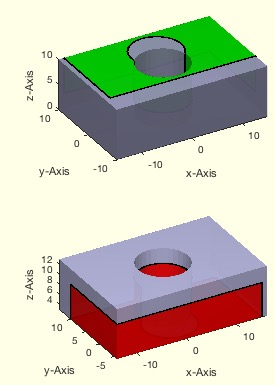% SGboolanalyze(A,B,thr) - plots the two separated surfaces of A and B when crossed% (by Tim Lueth, VLFL-Lib, 2017-AUG-14 as class: SURFACES)% % This fnctn show the principles of SGintersectFacetPoints, % VLFLinsertFacetPoints, SGisInterior and SGbool. (Status of: 2017-08-14)% % Introduced first in SolidGeometry 4.1% % See also: SGintersectFacetPoints, VLFLinsertFacetPoints, SGisInterior, % SGbool% % SGboolanalyze(A,B,[thr])% === INPUT PARAMETERS ===% A: Solid A; or number for CSGsample% B: Solid B; % thr: % % EXAMPLE: % SGboolanalyze(8)% % See also: SGintersectFacetPoints, VLFLinsertFacetPoints, SGisInterior, % SGbool% % % Copyright 2017 Tim C. Lueth
###### eps2- returns the rounding limit for triangular or quadratic problems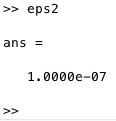% eps2 - returns the rounding limit for triangular or quadratic problems% (by Tim Lueth, VLFL-Lib, 2017-AUG-14 as class: AUXILIARY PROCEDURES)% % While fnctn eps returns the minimal distance between zero and a floting % points number, % eps 2 returns the root of this number. Therefor eps is a candidate to % round fnctns such as acos, cross, etc. (Status of: 2017-08-14)% % Introduced first in SolidGeometry 4.1% % See also: eps, roundn, acos, cross% % to=eps2% === OUTPUT RESULTS ======% to: rounding limit for square problems% % EXAMPLE: % eps2% VL=rand(100,3); VL=normr(VL); dp=bsxfun(@dot,VL',VL')'; % a=real(acos(dp)); [a roundz(a,eps2)]% % See also: eps, roundn, acos, cross% % % Copyright 2017 Tim C. Lueth
###### SGbooltest(tests)- Procedure to test different cases using SGbool and CSGsample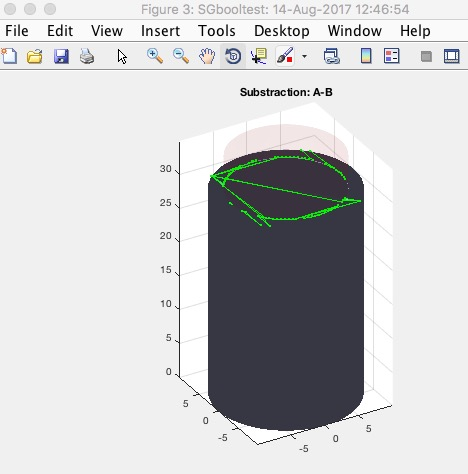###### VLiscollBBs(bba,VL)- returns the crossing of a vertex list with bounding boxes list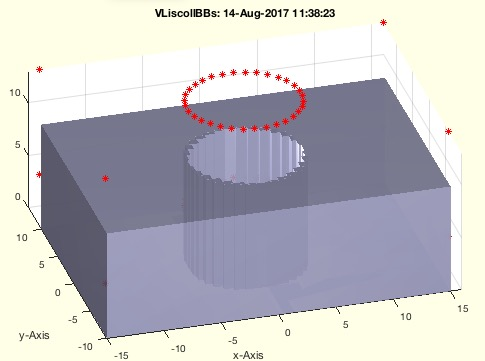% VLiscollBBs(bba,VL) - returns the crossing of a vertex list with bounding boxes list% (by Tim Lueth, VLFL-Lib, 2017-AUG-14 as class: ANALYZING PROCEDURES)% % This fnctn can be used directly to preprocess, i.e. accelerate facet % crossing fnctns (Status of: 2017-08-14)% % Introduced first in SolidGeometry 4.1% % See also: VLDLBBofVLFL, collofBBs% % [CL,ca,cb]=VLiscollBBs(bba,VL)% === INPUT PARAMETERS ===% bba: bounding box list A or Solid Geometry% VL: Vertex list to test% === OUTPUT RESULTS ======% CL: Crossing list [n x 2] = [ia ib]% ca: A% cb: optional index list of crossings of VL% % EXAMPLE: % [A,B]=CSGsample(5); VLiscollBBs(A,B.VL)% [A,B]=CSGsample(5); VLiscollBBs(A,A.VL)% % See also: VLDLBBofVLFL, collofBBs% % % Copyright 2017 Tim C. Lueth
###### VLFLfreeboundaryfacets(VL,FL,thr)- returns the facets attached to the freeboundary edges% VLFLfreeboundaryfacets(VL,FL,thr) - returns the facets attached to the freeboundary edges% (by Tim Lueth, VLFL-Lib, 2017-AUG-14 as class: SURFACES)% % During testing of SGbool it became obvious, that the result of SGbool % without a postprocessing will create structures that MATLAB's % triangulation procedure will consider as open edge structure while % Meshlab would consider it as perfect solid.% Those tests where performed using % 1. A 1e-12 grid on both solids!% 2. Non rounding during SGintersectFacetPoints% 3. Thresholds of 1e-6 for VL and 1e-9 for NPLs% 4. Thresholds for 1e-6 for UVP and added triangulation points% 5. Thresholds of 1e-6 for minimal area of a facet (line type) % Even than, there are problems with surface that come from the fnctn % SGisinterior, since there is a bug for coordinates ontop of barycentric % coordinates% A final fusion of 1e-8 on the point position solves this problem.% Therefore Meshlab roundes at least at 1e-8 / and the accuracy of SGbool % is maximum 1e-8;% % (Status of: 2017-08-15)% % Introduced first in SolidGeometry 4.1% % See also: VLFLplotfreeboundary% % [FL,EL]=VLFLfreeboundaryfacets(VL,FL,[thr])% === INPUT PARAMETERS ===% VL: Vertex list% FL: Facet list% thr: threshold for VLFLshort2; default is 0;% === OUTPUT RESULTS ======% FL: facets attached to the freeboundary edges% EL: freeboundary edges% % See also: VLFLplotfreeboundary% % % Copyright 2017 Tim C. Lueth
###### SGinsertJoint(SG,T,typ,x)- creates joint structures inside of a solid geometry% SGinsertJoint(SG,T,typ,x) - creates joint structures inside of a solid geometry% (by Tim Lueth, VLFL-Lib, 2017-AUG-13 as class: SURFACES)% % Introduced first in SolidGeometry 4.1% % SGR=SGinsertJoint(SG,T,typ,x)% === INPUT PARAMETERS ===% SG: Solid Geometry% T: Frame for the Solid Geometry% typ: Type of joint% x: parameter set% === OUTPUT RESULTS ======% SGR: % % EXAMPLE: % SG=SGsample(29); SGinsertJoint(SG,TofR(rot(0,0,0),[0 +20 5]));% % % Copyright 2017 Tim C. Lueth
###### VLFLplotfreeboundary(VL,FL,c,w)- plots the freeboundary of a solid with grid forced vertices (1e-6)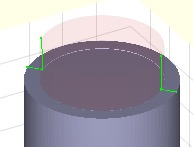% VLFLplotfreeboundary(VL,FL,c,w) - plots the freeboundary of a solid with grid forced vertices (1e-6)% (by Tim Lueth, VLFL-Lib, 2017-AUG-13 as class: USER INTERFACE)% % Points that have coordinate differences of less than 1e-6 are mapped to % the same vertices in the triangulation class. Therefor the threshold in % this fnctn is 1e-6; (Status of: 2017-08-15)% % Introduced first in SolidGeometry 4.1% % See also: VLFLfreeboundary, VLFLplots, VLELplot, VLFLplot, % VLFLfreeboundaryfacets% % h=VLFLplotfreeboundary(VL,FL,[c,w])% === INPUT PARAMETERS ===% VL: Vertex list% FL: Facet list% c: color; default is 'r-'% w: width of lines% === OUTPUT RESULTS ======% h: handle to the drawings% % EXAMPLE: % [A,B]=CSGsample(8); SGbool('A',A,B); X=ans; % VLFLplotfreeboundary(X.VL,X.FL);% % See also: VLFLfreeboundary, VLFLplots, VLELplot, VLFLplot, % VLFLfreeboundaryfacets% % % Copyright 2017 Tim C. Lueth
###### roundz(x,thr)- returns zeros if a number is larger than -thr and smaller than thr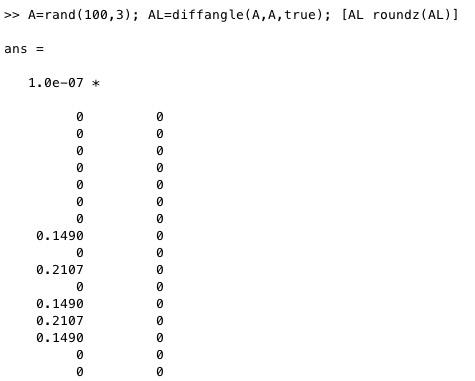% roundz(x,thr) - returns zeros if a number is larger than -thr and smaller than thr% (by Tim Lueth, VLFL-Lib, 2017-AUG-12 as class: AUXILIARY PROCEDURES)% % =======================================================================% OBSOLETE (2017-08-12) - USE FAMILY 'roundn' INSTEAD% =======================================================================% % roundn is twice as fast as roundz! Therefore use roundn% At lot of trigonometric fnctn and cross product or dot product have % problems with numerical accuracy.% Double = rounds(1,12)% single = rounds(1,6)% half = rounds(1,3) (Status of: 2017-08-12)% % Introduced first in SolidGeometry 4.1% % See also: [ roundn ] ; round, roundn% % y=roundz(x,[thr])% === INPUT PARAMETERS ===% x: floating point number% thr: threshold% === OUTPUT RESULTS ======% y: small numbers are set to zero% % EXAMPLE: % VL=rand(100,3); VL=normr(VL); dp=bsxfun(@dot,VL',VL')'; % a=real(acos(dp)); [a roundz(a,1e-6)]% % See also: [ roundn ] ; round, roundn% % % Copyright 2017 Tim C. Lueth
###### rounds(x,s)- is just an abbreviation if rounds(x,s,'significant')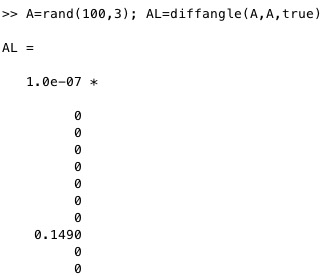% rounds(x,s) - is just an abbreviation if rounds(x,s,'significant')% (by Tim Lueth, VLFL-Lib, 2017-AUG-12 as class: AUXILIARY PROCEDURES)% % At lot of trigonometric fnctn and cross product or dot product have % problems with numerical accuracy.% Double = rounds(1,12)% single = rounds(1,6)% half = rounds(1,3) (Status of: 2017-08-12)% % Introduced first in SolidGeometry 4.1% % See also: round, roundn% % y=rounds(x,s)% === INPUT PARAMETERS ===% x: floating point number% s: number of % === OUTPUT RESULTS ======% y: rounded x% % See also: round, roundn% % % Copyright 2017 Tim C. Lueth
###### crosspointsVLFLofT(VL,FL,T,)- returns the crossing points of the axis of a coordinate system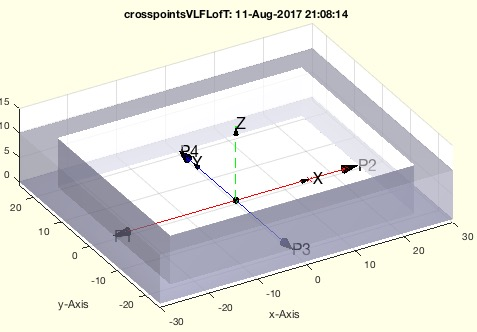% crosspointsVLFLofT(VL,FL,T,) - returns the crossing points of the axis of a coordinate system % (by Tim Lueth, VLFL-Lib, 2017-AUG-11 as class: KINEMATICS AND FRAMES)% % For a rotational axis, typically the z values TVL(5:6,:) are important% For a prismatic axis, typically the x axis TVL(1:2) is important% (Status of: 2017-08-12)% % Introduced first in SolidGeometry 4.1% % See also: crosspointVLFL, CVLofSGcutplanes, CVLofconvexHull% % [TVL,td,np,TN]=crosspointsVLFLofT(VL,FL,T,[])% === INPUT PARAMETERS ===% VL: Vertex List of Solid% FL: Facet List of Solid% T: Coordinate Frame [4x4]% === PROPERTY NAMES =====% 'center' : if 'center' is used; the Matrix TN contains the moved frame % matrix% === OUTPUT RESULTS ======% TVL: Touch vertex list [6 x 3]% td: distances between touch points [dx dy dz]% np: center of crossing lines% TN: Optional moved Frame matrix% % EXAMPLE: % VL,FL]=SGsample(5);crosspointsVLFLofT(VL,FL,eye(4))% VL,FL]=SGsample(29);% crosspointsVLFLofT(VL,FL,TofR(rot(0,0,0),[0 22 5]))% crosspointsVLFLofT(VL,FL,TofR(rot(0,pi/4,0),[0 22 5]))% % See also: crosspointVLFL, CVLofSGcutplanes, CVLofconvexHull% % % Copyright 2017 Tim C. Lueth
###### CVLofSGcutplanes(SG,T)- returns the three cutting contours at a frame coordinate system through a solid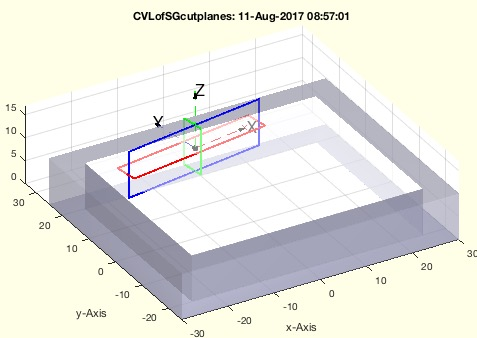% CVLofSGcutplanes(SG,T) - returns the three cutting contours at a frame coordinate system through a solid% (by Tim Lueth, VLFL-Lib, 2017-AUG-11 as class: EXPERIMENTS)% % Thus fnctn helps to create cutting contours for a solid using an % optional cutting frame coordinate system. In contrast to % CVLofconvexHull, this fnctn create a local cut that is only considering % the solid space around the frame coordinate system (Status of: % 2017-08-11)% % Introduced first in SolidGeometry 4.1% % See also: CVLofconvexHull, CVLofSGcutT, CVLofSGT% % [CVLX,CVLY,CVLZ,SG2]=CVLofSGcutplanes(SG,T)% === INPUT PARAMETERS ===% SG: Solid geoemtry% T: cutting coordinate system; must be inside of the solid!% === OUTPUT RESULTS ======% CVLX: cutting contour x=0 (green)% CVLY: cutting contour y=0 (blue)% CVLZ: cutting contour z=0 (red)% SG2: used T matrix; if input parameter T was empty% % EXAMPLE: % CVLofSGcutplanes(SGsample(29))% CVLofSGcutplanes(SGsample(30))% CVLofSGcutplanes(SGsample(29),TofP([0 22 5]))% CVLofSGcutplanes(SGsample(29),TofR(rot(pi/20,pi/20,pi/20),[0 22 5]))% % See also: CVLofconvexHull, CVLofSGcutT, CVLofSGT% % % Copyright 2017 Tim C. Lueth
###### CVLofconvexHull(SG,T)- returns the three cutting contours through the convex hull of a solid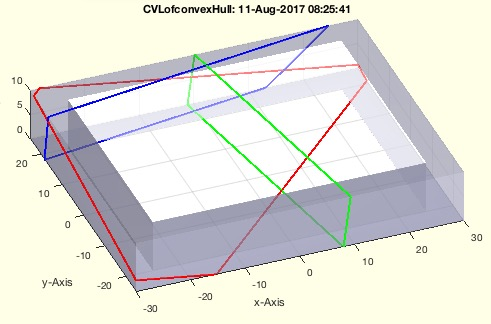% CVLofconvexHull(SG,T) - returns the three cutting contours through the convex hull of a solid% (by Tim Lueth, VLFL-Lib, 2017-AUG-11 as class: EXPERIMENTS)% % Thus fnctn helps to create cutting contours for a solid using an % optional cutting frame coordinate system (Status of: 2017-08-11)% % Introduced first in SolidGeometry 4.1% % [CVLX,CVLY,CVLZ,T]=CVLofconvexHull(SG,[T])% === INPUT PARAMETERS ===% SG: Solid geoemtry% T: cutting coordinate system; default is eye(4) at center of solid% === OUTPUT RESULTS ======% CVLX: cutting contour x=0 (green)% CVLY: cutting contour y=0 (blue)% CVLZ: cutting contour z=0 (red)% T: used T matrix; if input parameter T was empty% % EXAMPLE: % CVLofconvexHull(SGsample(29))% CVLofconvexHull(SGsample(30))% CVLofconvexHull(SGsample(29),TofP([0 22 5]))% CVLofconvexHull(SGsample(29),TofR(rot(pi/20,pi/20,pi/20),[0 22 5]))% % % Copyright 2017 Tim C. Lueth
###### textVLFS(VL,FL,FSi,c,s,nt,lb)- plots descriptors for each feature surface and the corresponding feature edges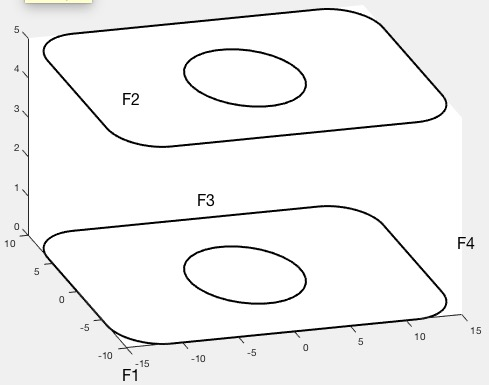% textVLFS(VL,FL,FSi,c,s,nt,lb) - plots descriptors for each feature surface and the corresponding feature edges% (by Tim Lueth, VLFL-Lib, 2017-AUG-10 as class: VISUALIZATION)% % This is an auxiliary fnctn to the boundaries of feature surfaces and % the feature surface descriptors% In contrast to FSplot, the surfaces itself are not drawn (Status of: % 2017-08-10)% % Introduced first in SolidGeometry 4.1% % See also: FSplot, textT, textP, textVL, textCVL, textVLFL, % VLFLfaceNormalplot% % h=textVLFS(VL,FL,FSi,[c,s,nt,lb])% === INPUT PARAMETERS ===% VL: Vertex list% FL: Facet List% FSi: Feature surface index list; FSi = surfacesofSG(SG,fea);% c: Color, default is black% s: Size, default is 10% nt: optional list of selected points% lb: optional string for text; default is 'F'% === OUTPUT RESULTS ======% h: handle to modify or delete text if necessary% % EXAMPLE: % close all; SG=SGsample(27); fea=0.08; FSi=surfacesofSG(SG,fea); % SGfigure; view(-30,30); h=SGplot(SG); setplotlight(h,'w',0.9);% textVLFS(SG.VL,SG.FL,FSi);% % See also: FSplot, textT, textP, textVL, textCVL, textVLFL, % VLFLfaceNormalplot% % % Copyright 2017 Tim C. Lueth
###### TofFS(TR,Nr,fe,FS,Rz,dz)- returns a HT matrix for a speficied feature surface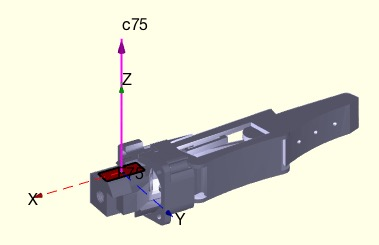% TofFS(TR,Nr,fe,FS,Rz,dz) - returns a HT matrix for a speficied feature surface% TofFS(TR,Nr,fe,FS,Rz,dz) - returns a HT matrix for a speficied feature surface% (by Tim Lueth, VLFL-Lib, 2017-AUG-09 as class: KINEMATICS AND FRAMES)% % This very powerful fnctn is similar to SGTui but creates Frames based % on Feature surfaces. The use is to specify the number of the feature % edg plus an optional specific feature selector such as% Feature Surface 3 based on an feature edge angle of 1 rad (60 degree).% Feature Surface 3 based on an feature edge angle of 1 rad (60 degree) % and here the third radius % It is also possible to eplace the feature angle directly by the FIL % list of surfacesof SG (Status of: 2017-08-10)% % Introduced first in SolidGeometry 4.1% % See also: SGTget, SGTset, SGTplot, SGTremove, SGTui, VLFLsurfaceofSGT, % FSplot% % [T,fsi,FN,Ti,RCVL]=TofFS([TR,Nr,fe,FS,Rz,dz])% === INPUT PARAMETERS ===% TR: Solid Geometry% Nr: Number list or ez vector list of the selected surfaces% fe: feature edge angle; default 1; use 0.08 for planar surfaces ~ 6 % degree% FS: Optional string selector for center points such as 'R1'% Rz: Optional Rz rotation angle% dz: Optional dz translation in % === OUTPUT RESULTS ======% T: Frame Matrix% fsi: feature surface index; scalar if unique or selected% FN: full feature name list% Ti: full frame list for feature names% RCVL: CVL for found radial contours; sorted% % EXAMPLE: % TofFS(SGsample(27),2)% TofFS(SGsample(27),2,'','R1')% TofFS(SGsample(27),4,'','R2')% TofFS(SGsample(27),76,0.05)% FIL=surfacesofSG(SGsample(27),0.05); TofFS(SGsample(27),76,FIL)% TofFS(SGsample(26,[0 0 1;0 -1 0])% % See also: SGTget, SGTset, SGTplot, SGTremove, SGTui, VLFLsurfaceofSGT, % FSplot% % % Copyright 2017 Tim C. Lueth
###### CVLofSGcutT(SG,T)- returns the CVL of a crossing/slicing plane given by a HT or Frame string% CVLofSGcutT(SG,T) - returns the CVL of a crossing/slicing plane given by a HT or Frame string% (by Tim Lueth, VLFL-Lib, 2017-AUG-09 as class: SURFACES)% % CVLofSGcutT uses SGslice3 for creating the cross sectional plane. In % constrast to CVLofSGT is crosses the complete solid not only the frame % surface (Status of: 2017-08-09)% % Introduced first in SolidGeometry 4.1% % See also: CVLofSGT% % [CVL,CPL]=CVLofSGcutT(SG,T)% === INPUT PARAMETERS ===% SG: Solid Geometry with Frames% T: 4x4 matrix or Frame name% === OUTPUT RESULTS ======% CVL: Convtour Vertex List% CPL: Closed contour wrt Frame N; [n x 3]; z should be 0% % EXAMPLE: loadweb JACO_robot.mat% CVLofSGcutT(JC1,'F');% % See also: CVLofSGT% % % Copyright 2017 Tim C. Lueth
###### exp_2017_08_08- Experiment to create a cylindrical link into any solid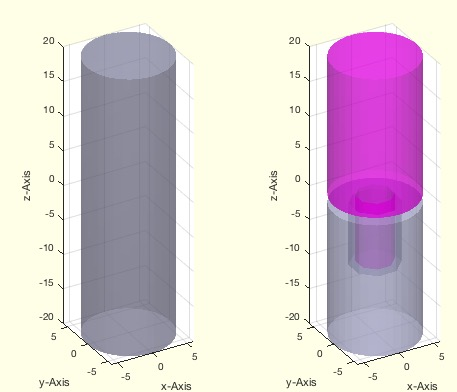% exp_2017_08_08 - Experiment to create a cylindrical link into any solid% (by Tim Lueth, VLFL-Lib, 2017-AUG-08)% % Using this Strategy it is possible to create any type of joint at a % specific position or Frame into a solid geoemtry. In other words, there % is obviously no need to create a joint before since the joint can be % created whenever and whenever desired. (Status of: 2017-08-08)% % Introduced first in SolidGeometry 4.1% % exp_2017_08_08% % % Copyright 2017 Tim C. Lueth
###### SGretesselateAmin(SG,amin)- retesselates a solid that all facet have a minimal size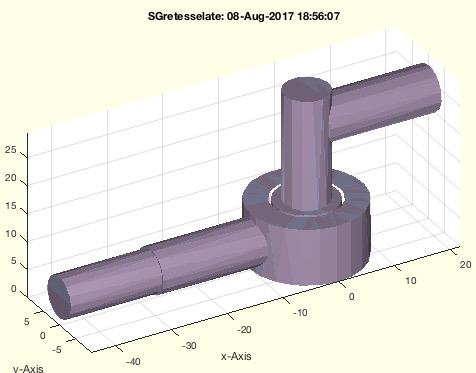% SGretesselateAmin(SG,amin) - retesselates a solid that all facet have a minimal size% (by Tim Lueth, VLFL-Lib, 2017-AUG-08 as class: SURFACES)% % This is a recursive fnctn that is repeated until all small facets are % removed.% Important fnctn that makes sense after SGbool (Status of: 2017-08-24)% % Introduced first in SolidGeometry 4.1% % See also: nofVLFL, SGreduceVLFL, SGbool, SGretesselate, SGdelaunay% % [SGR,nn,no]=SGretesselateAmin(SG,[amin])% === INPUT PARAMETERS ===% SG: Solid Geoemtry% amin: minimal facet size; default is 0.1mm^2% === OUTPUT RESULTS ======% SGR: Solid Geoemtry with no Facet smaller that amin% nn: final facets% no: original facets% % EXAMPLE: % SGretesselate(SGsample(27))% SGretesselate(SGsample(20),5)% % See also: nofVLFL, SGreduceVLFL, SGbool, SGretesselate, SGdelaunay% % % Copyright 2017 Tim C. Lueth
###### nofVLFL(VL,FL,amin);- returns the number of facets with an area larger than 0.1 mm^2 and the facet list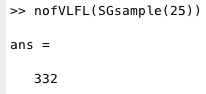% nofVLFL(VL,FL,amin); - returns the number of facets with an area larger than 0.1 mm^2 and the facet list% (by Tim Lueth, VLFL-Lib, 2017-AUG-08 as class: SURFACES)% % This fnctn is useful in combination with reducepatch/SGreduceVLFL after % an SG boolean operation. In such a case a lot of additional triangles % are added that could be removed by retesselation/% % (Status of: 2017-08-08)% % Introduced first in SolidGeometry 4.1% % See also: VLFLarea, SGreduceVLFL, reducepatch, SGfacenum% % [nf,Ai]=nofVLFL(VL,FL,[amin]);% === INPUT PARAMETERS ===% VL: Vertex list% FL: Facet list% amin: threshold; default is 0.1 mm^2% === OUTPUT RESULTS ======% nf: number of facets >= amin% Ai: Index list of facets >% % EXAMPLE: % nofVLFL(SGsample(17))% nofVLFL(SGsample(26))% % See also: VLFLarea, SGreduceVLFL, reducepatch, SGfacenum% % % Copyright 2017 Tim C. Lueth
###### SGfacenum(SG)- returns the number of facets of a solid geoemtry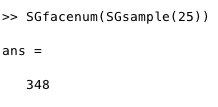% SGfacenum(SG) - returns the number of facets of a solid geoemtry% (by Tim Lueth, VLFL-Lib, 2017-AUG-08 as class: SURFACES)% % It makes sense to reduce the number of facets after an boolean melting % operation to the number of facets the object had before (Status of: % 2017-08-08)% % Introduced first in SolidGeometry 4.1% % See also: SGreduceVLFL, nofVLFL% % nF=SGfacenum(SG)% === INPUT PARAMETERS ===% SG: Solid Geometry, can be nested% === OUTPUT RESULTS ======% nF: number of facets% % EXAMPLE: % SGfacenum(SGsample(25))% % See also: SGreduceVLFL, nofVLFL% % % Copyright 2017 Tim C. Lueth
###### SGsurfacemeltbool(SG,si,Amin,grw)- returns a solid which surfaces were added using SGbool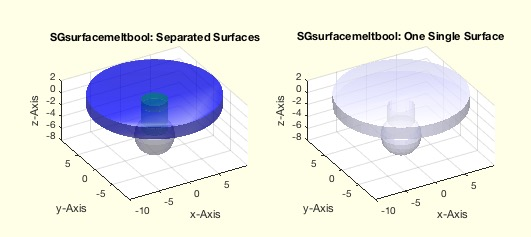% SGsurfacemeltbool(SG,si,Amin,grw) - returns a solid which surfaces were added using SGbool% (by Tim Lueth, VLFL-Lib, 2017-AUG-08 as class: SURFACES)% % This fnctn is useful to melt overlapping solids into one solid by using % SGboolTL (Status of: 2017-08-16)% % Introduced first in SolidGeometry 4.1% % See also: SGsurfaces, SGplotsurfaces, SGboolTL% % A=SGsurfacemeltbool(SG,[si,Amin,grw])% === INPUT PARAMETERS ===% SG: Original solid. All surfaces must overlap% si: indices of surfaces to melt; default is all;% Amin: Minimal Area removal; parameter for SGboolTL; default 0.01% grw: Minimal grow factor; parameter for SGboolTL; default 1e-5% === OUTPUT RESULTS ======% A: Melted Object consisting of melted solids% % EXAMPLE: % A=SGsample(27); B=SGaddrelSG(SGsample(5),A,'incenter','ontop',-1); % SGsurfacemeltbool(B)% SGsurfacemeltbool(SGsample(17),[3,2,5,6])% % % See also: SGsurfaces, SGplotsurfaces, SGboolTL% % % Copyright 2017 Tim C. Lueth
###### SGbool5(flag,A,B,thr)- returns the correct result of a boolean operation on two closed elementary solids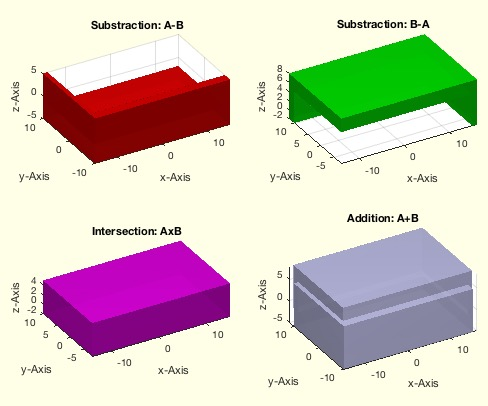% SGbool5(flag,A,B,thr) - returns the correct result of a boolean operation on two closed elementary solids% (by Tim Lueth, VLFL-Lib, 2017-AUG-08 as class: SURFACES)% % SGbool5 hast much less limitations than SGbool3 and SGBool4. All fnctns % SGbool3-5 are now much faster.% Use CSGsample for testing. % Nevertheless, it should be clear that there are limitations even of % closed surface models with SGbool3 and SGBool4: % 1. Never have identical points in both surfaces% 2. Never have identical lines in both surfaces% 3. Never have indentical surface planes in both surfaces% If there is a risc, use SGgrow to grow before substraction or addition % by 1e-4% It is possible to remove those problems one day by cutting tetrahedrons % instead of triangles% A => A without B% B => B without A% + => A combined with B% x => A intersected with B% % BTW: CATIA LIMITS THE SPATIAL RESOLUTION TO 0.01mm, ie. 1e-2 (Status % of: 2017-08-08)% % Introduced first in SolidGeometry 4.1% % See also: SGbool, SGbool2, SGbool3, SGbool4% % SGR=SGbool5(flag,A,B,[thr])% === INPUT PARAMETERS ===% flag: Boolean operator ('AB+x')% A: Solid A (VL/FL)% B: Solid B (VL/FL)% thr: threshold for grid; default is 1e-5% === OUTPUT RESULTS ======% SGR: resulting surface geometry% % EXAMPLE: % [A,B]=CSGsample(1); SGbool5('-',A,B);% [A,B]=CSGsample(2); SGbool5('-',A,B);% [A,B]=CSGsample(3); SGbool5('-',A,B); % [A,B]=CSGsample(4); SGbool5('-',A,B);% [A,B]=CSGsample(5); SGbool5('-',A,B);% [A,B]=CSGsample(6); SGbool5('-',A,B);% [A,B]=CSGsample(7); SGbool5('-',A,B);% % See also: SGbool, SGbool2, SGbool3, SGbool4% % % Copyright 2017 Tim C. Lueth
###### CSGsample(Nr,spatrel)- Cross-Pair Samples returns two solids as examples for crossing solids% CSGsample(Nr,spatrel) - Cross-Pair Samples returns two solids as examples for crossing solids% (by Tim Lueth, VLFL-Lib, 2017-AUG-06 as class: SURFACES)% % This is more or less an testing fnctn for SGbool and the supporting % fnctns:% SGintersectFacetPoints, VLFLinsertFacetPoints% The spatial relations are used from SGtransrelSG.% (Status of: 2018-07-28)% % Introduced first in SolidGeometry 4.1% % See also: CPLsample, SGsample, VLsample, PLsample, VLFLsample, % SGerrorsample, testfunctTL, permutevector% % [A,B]=CSGsample([Nr,spatrel])% === INPUT PARAMETERS ===% Nr: Nr of Example% spatrel: spatial relations such as 'ontop',-15% === OUTPUT RESULTS ======% A: Solid A% B: Solid A% % EXAMPLE: % CSGsample% [A,B]=CSGsample(3,'ontop',-15); SGintersectFacetPoints(A,B); NPL=ans,% VLFLinsertFacetPoints(B.VL,B.FL,NPL(:,[2 1 3:end])); copyfig; % VLFLinsertFacetPoints(A.VL,A.FL,NPL);% % See also: CPLsample, SGsample, VLsample, PLsample, VLFLsample, % SGerrorsample, testfunctTL, permutevector% % % Copyright 2017-2018 Tim C. Lueth
###### VLFLfaceNormalplot(VL,FL,c,t)- plots normal vector in appropriate length and writes the name of the facet at the tip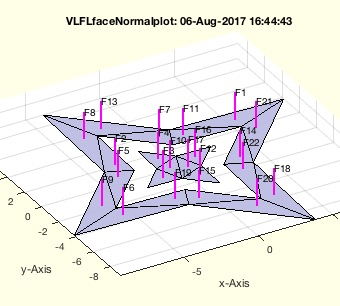% VLFLfaceNormalplot(VL,FL,c,t) - plots normal vector in appropriate length and writes the name of the facet at the tip% (by Tim Lueth, VLFL-Lib, 2017-AUG-06 as class: VISUALIZATION)% % plots only the normal vectors in appropriate length and writes the name % of the facet at the tip. Use VLFLplot(VL,FL) to show the solid itself. % (Status of: 2017-08-06)% % Introduced first in SolidGeometry 4.1% % See also: textT, textP, textVL, textCVL, textVLFL% % h=VLFLfaceNormalplot(VL,FL,[c,t])% === INPUT PARAMETERS ===% VL: Vertex list% FL: Facet List% c: Color, default is magenta% t: Thickness; default is 2% === OUTPUT RESULTS ======% h: handle to modify or delete text if necessary% % EXAMPLE: % [VL,FL]=VLFLsample(13); SGfigure; view(-30,30); VLFLplot(VL,FL); % VLFLfaceNormalplot(VL,FL);% % See also: textT, textP, textVL, textCVL, textVLFL% % % Copyright 2017 Tim C. Lueth
###### SGrotate(SG,ax,val)- returns a solid rotated around the center of the solid% SGrotate(SG,ax,val) - returns a solid rotated around the center of the solid% (by Tim Lueth, VLFL-Lib, 2017-AUG-06 as class: KINEMATICS AND FRAMES)% % Introduced first in SolidGeometry 4.1% % See also: SGtransT, SGTrotate% % SGN=SGrotate(SG,[ax,val])% === INPUT PARAMETERS ===% SG: Solid Geoemtry% ax: rotation axis sequence; 'xyz' or 'x' or 'z'% val: values for rotation; same as ax% === OUTPUT RESULTS ======% SGN: Spatial rotated Solid including the frames% % EXAMPLE: SGrotate(SGsample(18),'y',pi/2)% % See also: SGtransT, SGTrotate% % % Copyright 2017 Tim C. Lueth
###### workdir (wd)- changes to the working directory and/or defines it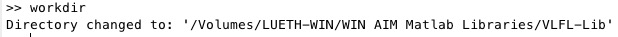% workdir (wd) - changes to the working directory and/or defines it% (by Tim Lueth, VLFL-Lib, 2017-AUG-04 as class: AUXILIARY PROCEDURES)% % Introduced first in SolidGeometry 4.1% % See also: desktopdir, pcodedirTL, smbFilename, smbPSLibname, expname% % workdir([wd])% === INPUT PARAMETERS ===% wd: working directory path% % See also: desktopdir, pcodedirTL, smbFilename, smbPSLibname, expname% % % Copyright 2017-2018 Tim C. Lueth
###### iscalledbycmdline- returns wether a function was called by commandline directly% iscalledbycmdline - returns wether a fnctn was called by commandline directly% (by Tim Lueth, VLFL-Lib, 2017-AUG-04 as class: AUXILIARY PROCEDURES)% % This is an auxiliary fnctn for developfnctns such as publishTL to % create help text in case that a fnctn is used not in a script or a % fnctn but in specific situations (Status of: 2017-08-04)% % Introduced first in SolidGeometry 4.1% % See also: SGtitle% % b=iscalledbycmdline% === OUTPUT RESULTS ======% b: logical true if length(dbstack) <=2% % See also: SGtitle% % % Copyright 2017 Tim C. Lueth